• CFD, Fluid Flow, FEA, Heat/Mass Transfer

Solver Setting

### Type of Solvers and Solution Control Parameters

This section deals with solution controls for solvers including topics like CFL Number, Time-step for Transient Simulations, Psuedo-time Marching, Parallel Computing, Nodes and Cluster, HPC - High Performance Computing, Threading, Partitioning, MPI - Message Passing Interface and Scalability.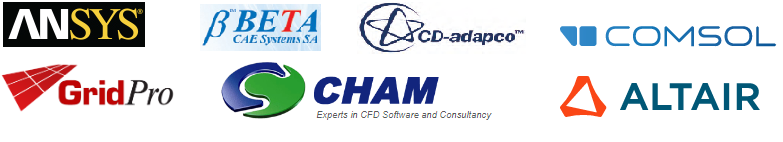Any program does only one thing: "Solves exactly what one tells it to solve and how to solve, nothing else!"

#### Solver setting

This activity of a CFD simulatin process involves selection of solver type (pressure-based or density-based, stead-state or transient), selection of [turbulence model, spatial discretization scheme (upwind, central difference), temporal discretization scheme (forward Euler, Backward Euler, Crank-Nicolson scheme, explicit leapfrog method), relaxation factor, PV-coupling method, transient time-step for solver (outer loop iteration), number of inner loop iterations for transient simulations, intermediate output data back-up frequency], gravity ON/OFF, creation of monitor points and monitor planes... The purpose of all these settings is to get a numerical scheme which is consistent, stable and convergent. Here stability refers to the property when errors caused by small perturbations in numerical method remains bound.

Note the the field variables solved during CFD simulation are velocity vector, pressure, temperature (enthalpy). The density at each cell is calculated from equation of state (ideal gas law or real gas laws like Redlich-Kwong and IAPWS equation of state for steam). Note that the "compressible flows" and "compressible gas" are not the same concepts. Compressible flows are characterized by Mach number of the flow whereas compressible behaviour of fluid refers to equation of state such as ideal gas law, essential change in density due to change in pressure and temperature.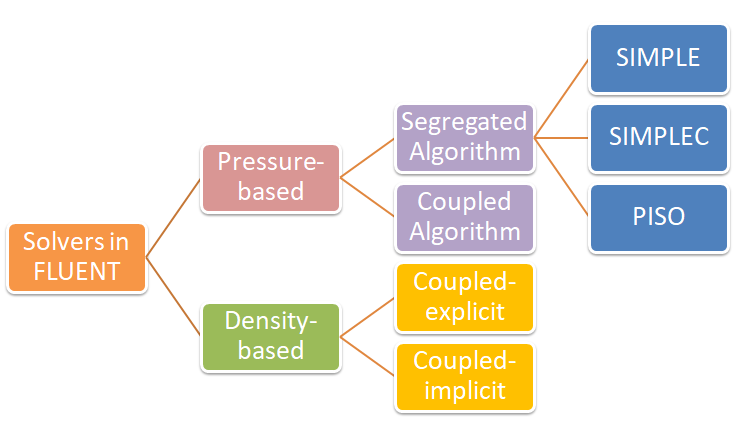Checklist of Solver Settings including Boundary Conditions

 No. Checkpoint Record 01 Has the basic checks been made: scale of mesh, quality, default interfaces settings (CFX may create unwanted interfaces)? 02 Has the density, viscosity and thermal conductivity of fluid been correctly assigned as per operating temperature and pressure? 03 Has the auto-save frequency and file name correctly defined? For runs on clusters, specify only file name without full path. 04 For transient simulations, have the specific heat capacity and density of solids been correctly assigned? 05 Has the relaxation factors for k, ε and turbulent viscosity been reduced to value lower than 1.0 say 0.25 or 0.50? 06 Has the convergence criteria been set to low value such as 1e-05 or lesser? Run may stop early if set to higher number such as 1e-3. 07 Has the discretization schemes set to first order for initial 500 ~ 1000 iterations? Gradually move to second order. 08 Has the monitor points been created for global mass imbalance? 09 In FLUENT, have contour plots been created? This helps avoid repeating the process while setting up different cases with same geometry. 10 Has a monitor for heat transfer through all walls been created? Do not include inlets and outlets. 11 Have the interfaces of porous and fluid domains been changed to internal? 12 Have the shadow walls checked and changed to coupled? Changing them to type Heat Flux or Temperature will decouple the two walls.

Notes:

• Segregated solvers are fast but less stable than coupled solvers. Segregated solvers is more likely to diverge even with low relaxation factors. Sometimes, the residuals (especially continuity and momentum) may not drop below certain value with segregated solver. Hence, change the P-V coupling to coupled solver and the residual levels drop significantly. Note that this may or may not result in significant improvement of field values (u, v, w and p).
• For multiple inlets and multiple outlets, it is better to use coupled (psuedo transient) solvers.
• Do not reduce under-relaxation for engegy less than 0.9. The convergence of energy equation slows down significantly.
• FLUENT creates shadow zones with extension :external for all walls on which shell conduction is enabled. This is available only for post-processing operations.
• The TUI option to set under-relaxation factor is different for coupled (solve set psuedo-relaxation-factor) and segegrated (solve set relaxation-factor) P-V-coupling in FLUENT.
• Pressure jump and fan boundary conditions can be used only for small values say 100 ~ 500 [Pa]. It cannot be used to model large pressure head such as 10 [kPa] with ideal gas.
• Switch OFF the default interface creation option in CFX. It will merge zero thickness walls into interior if fluid zones exists on the two sides.
• Refer to this page to get automation scripts and TUI commands.
• If you get high number of 'incomplete' trajectories of particles in DPM even with very high number of steps, the reason could be 'rebound' settings at the outlets.

#### Excerpts from user manuals of commercial tools

• Smaller physical timesteps are more robust than larger ones.
• An 'isothermal' or cold-flow simulation is more robust than modeling heat transfer. The Thermal Energy model is more robust than the Total Energy Model.
• Velocity or mass specified boundary conditions are more robust than pressure specified boundary conditions. Static pressure boundary is more robust than a total pressure boundary.
• If the characteristic time scale is not simply the advection time of the problem, there may be transient effects holding up convergence. Heat transfer or combustion processes may take a long time to convect through the domain or settle out. There may also be vortices caused by the initial guess, which take longer to move through the entire solution domain. In these cases, a larger timestep may be needed to push things through initially, followed by a smaller timestep to ensure convergence on the small time scale physics. If the large timestep results in solver instability, then a small time scale should be used and more iterations may be required.
• Sometimes the levels of turbulence in the domain can affect convergence. If the level of turbulence is non-physically too low, then the flow might be "too thin" and transient flow effects may be dominating. Conversely if the level of turbulence is non-physically too high then the flow might be "too thick" and cause unrealistic pressure changes in the domain. It is wise to look at the Eddy Viscosity and compare it to the dynamic (molecular) viscosity. Typically the Eddy Viscosity is of the order of 1000 times the dynamic viscosity, for a fully turbulent flow.
• The 2nd Order High Resolution advection scheme has the desirable property of giving 2nd order accurate gradient resolution while keeping solution variables physically bounded. However, may cause convergence problems for some cases due to the nonlinearity of the Beta value. If you are running High Res and are having convergence difficulty, try reducing your timestep. If you still have problems converging, try switching to a Specified Blend Factor of 0.75 and gradually increasing the Blend Factor to as close to 1.0 as possible.

Compatibility of several ANSYS FLUENT model with underlying physics and turbulence model are tabulate below (reference ANSYS FLUENT User's Guide)##### Compressibility of Fluids

One of the key consideration is the compressible or incompressible behaviour of the fluid. For liquids under lower pressure conditions (except cases such as hydraulics, deep bore drilling in oil exploration...), they can be assumed incompressible without loss of any accuracy. The compressibility chek for liquids need to be decided based on bulk modulus, operating pressures and coefficient of volume expansion.. The later varies between 0.001 ~ 0.010 [K-1]. The bulk modulus is defined as β = -V × ΔP/ΔV where negative sign refers to the fact that volume decreases as pressure increases. Thus, in terms of density, β = -ρ/Δρ × ΔP. β for water is 2.2 [GPa]. Hence, at pressure of 100 [MPa], the change in water density as compared to density at 1 [atm] would be 1000 [kg/m3] × 0.1 [GPa] / 2.2 [GPa] = 45.5 [kg/m3] which is 4.55% of density of water at 1 [atm].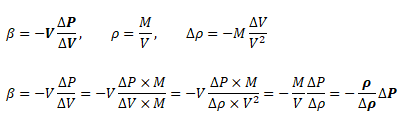While the change in density of liquids is more pronouced with change in temperature, the situation is different in cases of gases where density is a strong function of both temperature and pressure changes. Note that the density is calculated based on static conditions (static temperature and static pressure) and not on total conditions (total temperature and total pressure). On the other hand, the stagnation conditions are the true measure of pressure energy and internal energy. There are two modes of reduction in stagnation (or total) pressure: the first mode is due to conversion of pressure energy into velocity (kinetic) energy and the second mode is loss of pressure energy due to wall friction and minor losses (sudden expansion and contraction). The general equations to estimate stagnation and static conditions for all values of flow velocities are described below.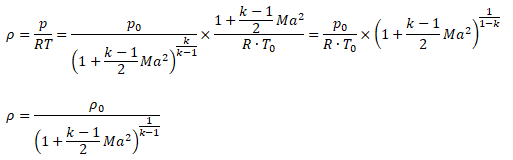Let's consider isothermal flow through a rough pipe of uniform cross-sectional area. The expression for frictional pressure drop is also described. Here, V denotes area-average velocity at the inlet and the outlet.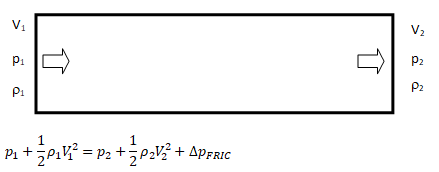From conservation of mass: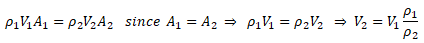Static pressure drop taking into account compressibiity of the fluid: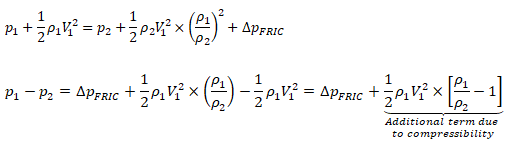Since ρ1 always > ρ2 for isothermal flows, the effect of compressibility of the gas is to increase static pressure drop. Since wall friction is also function of velocity gradient near wall and the free-stream velocity increases as density of gas decrease due to frictional loss, the effect of compressibility is to increase wall friction and subsequently viscous losses. But how much will the compressibility affect static pressure drop? For ρ1 / ρ2 = 1.11 (10% drop in density), V1 = 5 [m/s] and ρ1 = 1.20 [kg/m3], additional pressure drop due to compressibility = 0.5 × 1.20 × 25 × (1.11 - 1.00) = 0.66 [Pa]. However, a 10% reduction in density will call a 10% drop in absolute static pressure. At mean value of 1 [bar], this turns out to be 100,000 x 0.1 = 10,000 [Pa]. This magnitude of pressure drop can be observed in a pipe of diameter 100 [mm] and length of approximately 2.2 [km]!

The operating pressure is used to specify the mean pressure in the system. Though it can be adjusted to convert the gauge pressure into absolute pressure. That is, the operating pressure can be set to zero to get the pressure field directly in absolute values. This is the recommended method for cases involving phase change such as simulation of cavitation in pumps. Note that cavitation occurs when local absolute static pressure in the flow field falls below saturation pressure at given temperature. However, once the operating pressure is set to 0 [Pa], the boundary conditions should also be specified in absolute values, e.g. 1,01,325 [Pa] instead of 0 [Pa].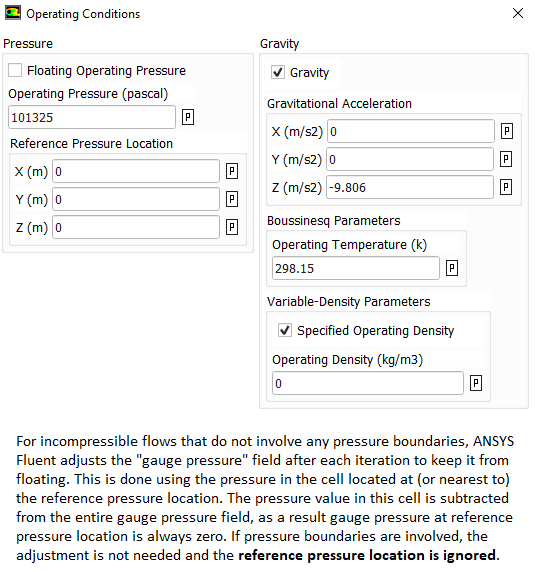In ANSYS Fluent, some fields are clearly marked with 'Gauge' to instruct users to specify the gauge pressures. However, this does not mean other pressure values (either user input or calculated in Fluent) are not gauge pressure. As per section 8.14.2 of User Guide: "All pressures that you specify and all pressures computed or reported by ANSYS FLUENT are gauge pressures." As per section 8.14.3: " Since ANSYS FLUENT always uses gauge pressure, you can simply set the operating pressure to zero, making gauge and absolute pressures equivalent." The reference pressure location is, by default, the cell center at or closest to (0,0,0). define -> operating-conditions -> used-ref-pressure-location: This text command is available only when the case is initialized and has no pressure-related boundary zones.
The solver-setting process encompasses following aspects of numerical solutions.
• Discretization scheme for momentum, pressure, energy and turbulence parameters: The PRESTO (Pressure Staggering Option) is recommended for pressure because in flows with high swirl numbers.
• PV-Coupling such as SIMPLE, SIMPLEC, SIMPLER, PISO
• Gradient schemes: Green-Gauss node based or Green-Gauss cell-based.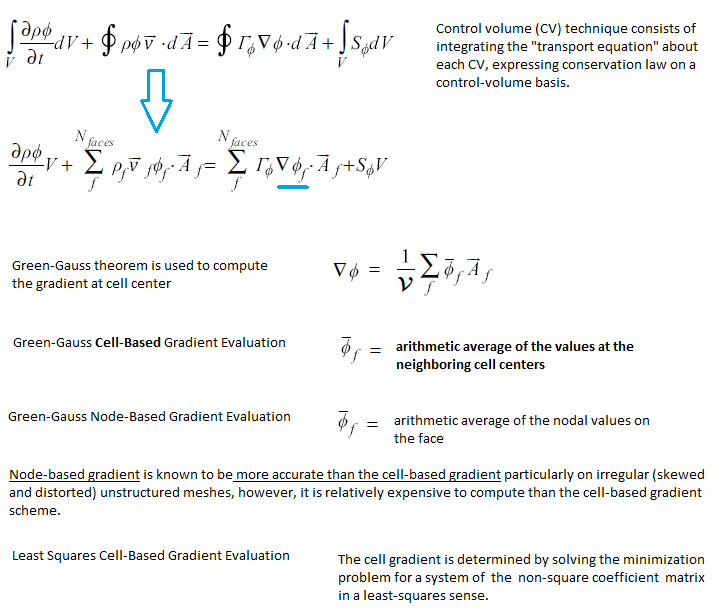On irregular (skewed and distorted) unstructured meshes, the accuracy of the least-squares gradient method is comparable to that of the node-based gradient (and both are much more superior compared to the cell-based gradient).
• Conservation target and residual levels for convergence criteria. In addition to the "residual norm", it is strongly recommended to set "mass-conservation" or "energy-conservation" as convergence check.
• Fluid time-scales
• Solid time-scales (in case of conjugate heat transfer or pure conduction problems). The recommended practice is to have solid time-scale set to an order of magnitude (10 times) higher than fluid time-scale.
• One should ensure that the solution is mesh-independent and use mesh adaption to modify the mesh or create additional meshes for the mesh-independence study. A mesh-sensitive result confirms presence of "False Diffusion".
• The node-based averaging scheme is known to be more accurate than the default cell-based scheme for unstructured meshes, most notably for triangular and tetrahedral meshes.
• Note that for coupled solvers which solves psuedo-transient equations even for steady state problems (such as CFX), relaxation factors are not required to be set. The solver applies a false timestep as the convergence process is iterated towards final solution.
• QUICK or Geo-Reconstruct scheme for the volume fraction in FLUENT

SIMPLE Algorithm: Analogy between analytical solution and numerical simulation#### Differences between collocated (non-staggered) and staggered grid layout• For collocated solvers (such as ANSYS CFX), control volumes are identical for all transport equations, continuity as well as momentum.
• CFX is a node-based solver and constructs control volumes around the nodes from element sectors, thus the number of control volumes is equal to the number of nodes and not the number of elements. The input of CFX may be tetrahedron, prism and hexhedron in physical form, the solver internally generates a polyhedral mesh. In a cell centered code, such as FLUENT or STAR-CD / STAR-CCM+, number of elements are the same as the number of control volumes (as the control volumes are same as physical elements) so these are often used interchangeably.
• Node-based Solvers: All field (or unknown or solution) variables as well as fluid properties are stored at the nodes (the vertices on the mesh).
• The distinction between collocated and staggered approach is nicely explained in section 4.6 by S. V. Patankar in his book "Numerical Heat Transfer and Fluid Flow" and section 7.2 in the book Computational Methods for Fluid Dynamics by J. H. Ferziger and M. Peric.
• For staggered (cell-based) solvers like ANSYS FLUENT, values of scalar field variables as well as material properties are stored as cell centres.
• Due to the difference in the way field variables are stored, the simulation with same mesh, material properties and boundary conditions, the y+ value reported in ANSYS CFX will be approximately twice that reported in FLUENT.

#### Compress Solution Files

The file size pf set-up files generated during CFD simulations is relatively large depending upon the number of cells and physics solved. Large files take time to upload/download and even compression-decompression is time consuming. However, compression is only feasible option to minimize issues related to data transfer. On Windows: while creating/splitting zip files using 7zip, there is option to choose the number of threads for parallel processing which can be used to ZIP the file or folder quickly. However, UNZIP operation inherently runs in serial mode. In Linux: "tar -cvzf zippedFolder.tar.gz f1.pdf f2.docx f3.msh dirReports" can be used to compress/tar files and folders together. However, it works in serial mode only.

Separating cells by Mark (Adaptation Register) /mesh/modify-zones/slit-face-zone 3 5 (): slit the faces of wall zones 3 and 5, either delete or deactivate the separated cell zone.

To mark and display bad cells, use "Cell Register" from model structure tree and then select 'Mesh' under "Field Value of" drop-down. This option is available only when fields are initialized.Cell Registers ➔ New ➔ Field Variable ➔ Type: Cells Less Than ➔ Field Value of: Skewness ➔ Click Save/Display

Alternatively, use Adapt Iso-value Mesh Select the property you want to adapt (e.g. Skewness or Orthogonal Quality in this case) Click 'Compute" to get the minimum and maximum value of the property in the domain Set the minimum and maximum bounds to select cells between these bounds. For example, you can set skewness with min 0.95 and max 1.0 to select all cells with a skewness bigger than 0.90 Click Adapt to remesh them.

#### Solver Setting: Physics

The flow regime and classification of laminar, transition and turbulent zones are unique to every flow. One of the most widely used flow condition is flow over a cylinder in cross flow. The following summary is an interesting description of how the flow evolve:
• Re ≤ 5, flow is laminar throughout and no vortices are present downstream of the body.
• 5 < Re < 40, a vortex pair can be observed in the wake region though flow is still laminar in the boundary layer.
• 40 ≤ Re ≤ 200, a von Karman vortex street is formed as where pairs of counter-rotating vortices are shed downstream of the body. The vortex shedding frequency is given by a non-dimensional Strouhal number: St = f × D / U.
• 200 ≤ Re ≤ 300, the flow behind a cylinder undergoes transition to a turbulent wake.
• 300 ≤ Re ≤ 3×105, the sub-critical Reynolds number regime wake is turbulent while the boundary layer is still laminar.
• Re > 3×105, a transition from laminar to turbulent boundary layer separation occurs.
In any practical application, the flow phenomena may not be laminar throughout or turbulent throughout. Hence, solver has to deal with different length scales and the result should be validated carefully. Following image describes the options availabe for physical model in STAR-CCM+.#### Solver Setting for Transient Simulation: CFL Number

One of the concern is to chose a right time-step value in transient simulation to have the best compromize between computational time and accuracy. Can a very very small time step be unconditionally stable? CFL (Courant–Friedrichs–Lewy) number is mathematically represented as Co = a.Δt/Δx. Here, a is the characteristic wave speed of the system. Why wave speed, there is no wave phenomena in the flow? It may not be apparent though the velocity field varies both in space and time domain - which is typical characteristics of wave propagation phenomena. The wave characterics is the reason Courant number is a measure of how much information 'u' traverses a cell or element of computational mesh (Δx) in a given time-step (Δt). For most use cases, 'a' can be assumed equal to the velocity scale while estimating Δt for a given Δx.

In an explicit Eulerian method, the Courant number cannot be > 1 otherwise it will lead a condition where information is propagating through more than one grid cell at each time step. This is explained in the physical domain of dependence and numerical domain of dependence in following diagram. For a stable solution, numerical domain of dependence must cover the physical (real) domain of dependence.In multi-dimensional problem, depending upon the spatial and temporal discretization scheme, the CFL condition varies as shown below.Thus, Courant number can be reduced either by reducing the time step or by increasing the mesh size (i.e. coarsening of the mesh). The later option may appear counter-intuitive though mathematically that remains a fact. It is difficult for most of the simulation engineers to believe the fact that coarsening the mesh help them achieve a better convergence.

• The CFL number scales the time-step sizes that are used for the time-marching scheme of the flow solver.
• A higher value leads to faster convergence but can lead to divergence and unstable simulations. The (stiff) CFL condition can be relaxed or softened by using implicit Euler such as Crank–Nicolson which is unconditionally stable.
• The inverse of this is also true where choosing a smaller value in an unstable simulation improves convergence.
• ANSYS FLUENT uses implicit (first order and second order) formulation for pressure-based solver. As per the user guide: "It is best to select the Coupled pressure-velocity coupling scheme if you are using large time steps to solve your transient flow, or if you have a poor quality mesh."
• In general, the solution at the end of (inner iterations in) current time-step is used as initial value for iterations at next time-step. ANSYS FLUENT provides option to extrapolate the solution variable values for the next time step using a Taylor series expansion. This can improve the convergence of the transient calculations by enabling and the absolute residual levels are lowered during inner iterations.
So how should one chose the best time step, Δt? There is not unique option and need few trials at the early stage of transient phenomena. A recommended method is to calculate starting value of Δt = Δx/V/10 where Δx is the minimum grid spacing in computational grid. Observe the number of inner iterations chosen solve needs to converge at each time step. The ideal number of iterations per time step is 5 to 10. If the solver needs substantially more number of time steps, the Δt is too large to capture the transient effect. If the solver takes only a few iterations per time step, Δt is unnecessarily small and can be increased without loss of accuracy or likelihood of convergence issues.

To verify that your choice for time-step was proper, check contours of the Courant number within the domain. In FLUENT, select Cell Courant Number under Velocity... For a stable and accurate calculation, the Courant number should not exceed a value of 20-40 in most sensitive transient regions of the domain.

Note that CFX reports two types of Courant number: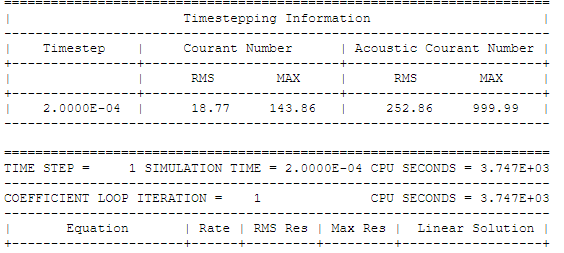#### Material Properties

Sometime underlying physics and type of solver chosen governs the material definition. For example, a density based solver requires density to be function of pressure and temperature. Similarly, simulation of Joule-Thomson phenomena (cooling effect due to throttling) requires material properties to be modeled as real-gas. An ideal gas will never cause this effect. The simulation related to steams required careful understanding of state of steam: wet-steam vs. dry steam. An incompressible flow may required only density and viscosity of a fluid whereas phase change and/or reacting mixture phenomena will require definition of reference enthalpy and entropy.

Similarly, air can be treated as 4 different ways depending upon application such as:

 Air for common applications Air in HVAC and Psychrometrics Air in Hydrocarbon Fuel Combustion Air in Pneumatic and High Pressure applications Ideal gas with moelcular weight = 28.96 [g/mole] Mixture of gases: Dry air + Water vapour Mixture of gases: Oxygen, Nitrogen, Water Vapour, Argon Real Gas with behaviour different from ideal gases

In some applications such as fans, there are significant reduction in pressure values (such as trailing edge and flow separation regions) as well as significant increase in pressure values (near leading edge). Hence, flow simulations dealing with gas and turbo-machine should use ideal gas law as final settings. Constant density can be used initially to get a convergence. The option of incompressible-ideal-gas available in ANSYS FLUENT makes the density a function of temperature only. This option also does not take into account change in density due to variation of pressure near the blade tip and separation regions.

To get a better accuracy, temperature dependent properties should be used. Some correlations available for gases are tabulated below. The Sutherland coefficients for some common gases are as follows. μ = μ0 * (273+C)/(T+C)*(T/273)1.5 where C is Sutherland coefficient in [K], T is fluid temperature in [K] and μ is viscosity in [Pa.s]. Reference: Handbook of Hydraulic Resistance by I. E. Idelchik.

 Fluid μ0 [Pa.s] C [K] Air 17.12 111 N2 16.60 104 O2 19.20 125 CO2 13.80 254 H2O-g 8.93 961 H2 8.40 71

The variation in dynamic viscosity of air in the temperature range 0 to 100 [°C] is of the order of 25% which is a significant amount if the wall friction contributes more to the pressure drop in the system. Similarly, a 5% reduction in local pressure shall cause 5% reduction in density and hence the velocity or volume flow rate has to increase by 5% to ensure the mass balance.

Specific Heat Capacity - Reference: B. G. Kyle, Chemical and Process Thermodynamics - Cp [kJ/kmol-K] ≡ [J/mol-K] = a + b.T + c.T2 + d.T3 where T is in [K]. For air, the coefficients are: a = 28.11 [kJ/kmol-K], b = 1.967E-03 [kJ/kmol-K2], c = 4.802E-06 [kJ/kmol-K3], d = -1.966E-09 [kJ/kmol-K4]. Taking molecular weight of air to be 28.96 [kg/kmol], the coefficients to calculate Cp in [J/kg-K] are: a = 970.65 [J/kg-K], b = 0.06792 [J/kg-K2], c = 1658E-04 [J/kg-K3], d = -6.788E-08 [J/kg-K4]. As you can notice, there is > 1% variation in specific heat capacity of air over the range of 100 [°C] and hence it can be assumed a constant value in calculations and simulations. Also note that for steady state simulations, the specific heat capacity and even density is not needed for solids. This can be inferred from the conduction equation for steady state conditions where only thermal conductivity appears as coefficient.

Thermal Conductivity - Reference: B. G. Kyle, Chemical and Process Thermodynamics - k [W/m-K] = a + b.T + c.T2 + d.T3 where T is in [K]. a = -3.933E-04 [W/m-K], b = 1.018E-04 [W/m-K2], c = -4.857E-08 [W/m-K3], d = 1.521E-11 [W/m-K4]. There is 25% increase in thermal conductivity or air in the temperature range 15 ~ 100 [°C] and hence a constant thermal conductivity may yield lower heat transfer rate.

Non-Newtonian Fluids: Most of the fluids behave such that shear stress is linear function of strain rate with zero initial threshold value of shear stress. However, there are some material where shear stress is non-linear function of strain rate with non-zero initial threshold value of shear stress (yield point) before which flow cannot start. Various type of such material also known as Bingham plastic and Herschel-Bulkley are described below.The standard enthalpy of formation of a material or compound is defined as the change in enthalpy (ΔH0) when 1 mole of that material in the standard state (1 atm of pressure and 298.15 K) is formed from its pure elements under the same temperature and pressure conditions. The standard enthalpy of formation of a pure element in its reference is zero. Carbon exists as C-atoms, oxygen exists as O2 molecules and hydrogen exists as H2 in its reference (or natural) form. Hence, the standard enthalpy of formation is ZERO. However, water-vapour and carbon-dioxide are not pure elements and will have non-zero value as their standard enthalpy of formation.

The value of ΔH0 will be negative if the process is endothermic (that is the reaction for material formation requires external heat energy) or positive if the process is exothermic (if heat is released during formation or reaction).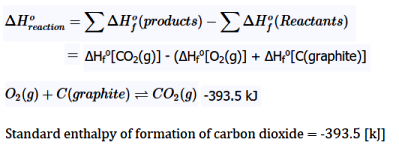In situations with phase change such as forced convection subcooled nucleate boiling, the standard state enthalpies of vapor and liquid phase are set such that their difference equals to latent heat of vapourization = 2.992E+07 [J/kmole]. The unit of standard state enthalpy is [kJ/kmol] and usually the latent heat value is avaiable in [kJ/kg]. The latest heat value in [kJ/mole] should be multiplied by molecular wight of the liquid. For correct specification of latent heat, the reference temperature should be set to 298.15 [K] or 25 [°C]. Note that it is a positive value (in case of boiling simulation) as compared to standard state enthalpies of species such as CO2 and CH4 (which are negative by sign convection) used in species transport and combustion modeling.

In phase change phenomena (evaporation and/or condensation), the difference between actual temperature of the phase and saturation conditions are described as degree of subcooling (liquid) and degree of superheating (gas). If the inlet temperature of liquid is T = 350 [K] and the saturation temperature TSAT = 373 [K], liquid state is terms as subcooled and with the degree of subcooling = 373 - 350 = 23 [K]. In a nucleate boiling, when the wall temperature rises above the liquid saturation temperature, steam bubbles will get formed and migrate away from the wall. Since the bulk flow (liquid far away from the wall) is subcooled, these bubbles condense near the center of the pipe.

Wet Steam: The steam with droplets of water is known as wet steam and it characterized by dryness fraction. Most of the boilers do not generate dry steam as steam bubbles breaking through the heated surface will pull tiny water droplets along with it. In general, when a substance exists partly as liquid and partly as vapor (gas) phase, its quality is defined as the ratio of the mass of vapor to the total mass. On the hand, mass fraction of the condensed phase is known as wetness factor.

Dry Steam: Saturated or superheated steam are known as dry steam.

For water, the triple point T = 0.01 [°C] and P = 0.6113 [kPa], is selected as the reference state where the internal energy, enthalpy and entropy of saturated liquid are assigned a zero value. Similarly, saturated refrigerant R-134a is specified enthalpy of 0 [J/kg] at temperature -40 [°C] and pressure of 51.25 [kPa]. Thus, standard state enthalpy of liquid water is 0 and reference temperature is 273.15 [K]. The standard state enthalpy of steam is heat of vaporization at T = 0.01 [°C] and P = 0.6113 [kPa] and equals 2501 [kJ/kg]. The molecular weight of water is 18.0153 [kg/kmole] and hence the standard state enthalpy of steam is (2501 × 18.0153) [kJ/kmole]. The saturation pressure of water as per ASHRAE: p(T in [K]) in [Pa] = exp[c1 / T + c2 + c3 * T + c4 * T2 + c5 * T3 + c6 * Ln(T)] where c1 = -5800.22, c2 = +1.3914993E+00, c3 = -4.8640239E−02, c4 = +4.1764768E−05, c5 = −1.4452093E−08, c6 = +6.54597.

#### Porous Domains

Flow geometry such as heat exchangers with closely spaced fins, honeycomb flow passages in a catalytic converters, screens or perforated plates used as protection cover at the from of a tractor engine ... are too complicated to model as it is. They are simplified with equivalent performance characteristic, knowns as Δp-Q curve. These curves are either generated using empirical correlations from textbooks or using a CFD simulation for smalled, periodic / symmetric flow arrangement. The simplified computational domain is known as "porous zone" in case it is represented as a 3D volume or pressure or porous jump in case it is represented as a plane of zero thickness. In a similar fashion, the performance data of a fan can be specified including the swirl component.

All the porous media formulation take the form: Δp = -L × (A.v + B.v2) where v is the 'superficial' flow velocity and negative sign refers to the fact that pressure decreases along the flow direction. The 'superficial velocity' is calculated assuming there is no blockage of the flow. L is the thickness of the porous domain in the direction of the flow. Here, A and B are coefficients of viscous and inertial resistances.

In FLUENT, the equation used is: Δp/L = -(μ/α.v + C2.ρ/2.v2) where α is known as 'permeability' and μ is the dynamic viscosity of fluid flowing through the porous domain. This (permeability) is a measure of flow resistance and has unit of [m2]. Other unit of measurement is the darcy [1 darcy = 0.987 μm2], named after the French scientist who discovered the phenomenon.

STAR-CCM+ uses the expression Δp/L = -(Pv.v + Pi.v2) for a porous domain.

The pressure drop is usually specified as Δp = ζ/2·ρ·v2 where ζ is 'equivalent loss coefficient' and is dimensionless. Darcy expressed the pressure gradient in the porous media as v = -[K/μ]·dP/dL where 'K' is the permeability and 'v' is the superficial velocity or the apparent velocity determined by dividing the flow rate by the cross-sectional area across which fluid is flowing.

Steps to find out viscous and inertial resistances:

1. Calculate the pressure drop vs. flow velocity data [Δp-v] from empirical correlations or wind-tunnel test or simplified CFD simulations.
2. Divide the pressure drop value with thickness of the porous domain. Let's name it as [Δp'-v curve].
3. Calculate the quadratic polynomial curve fit coefficients [A, B] from the curve Δp'-v. Ensure that the intercept to the y-axis is zero.
4. In STAR-CCM+ these coefficients 'A' and 'B' can be directly used as Pv and Pi which are viscous and inertial resistance coefficients respectively.
5. Divide 'A' by dynamics viscosity of the fluid to get inverse of permeability that is 1/α [m-2] to be used in ANSYS FLUENT as "viscous resistance coefficient" [m-1].
6. Divide 'B' by [0.5ρ] where ρ is the density of fluid, to get C2 to be used in ANSYS FLUENT as "inertial resistance coefficient".
7. The method needs to be repeated for the other 3 directions. If the flow is primarily one-directional, the resistances in other two directions need to be set to a very high value, typically 3 order of magnitude higher.
The GUI to set porous domain in ANSYS FLUENT is as shown below. For 3D cases, direction vectors for any two principal axes need to be specified, the third direction is automatically calculated by FLUENT. However, one must be consistent in specification of direction vector and resistance coefficients.In case porous domain is not aligned to any coordinate direction, the direction of unit vector along the flow and across the flow directions can be estimated from following Javascript program. Note that empty field is considered as 0.0. There is no check if a text value is specified in the input fields and the calculator will result in an error.

 First point - X1: First point - Y1: First point - Z1: Second point - X2: Second point - Y2: Second point - Z2:

Temperature Drop across a Porous Domain

If flow through the porous domain is assumed (a) incompressible, one-dimension, steady state and (b) pressure drop varies linearly with the flow direction x:##### Named Expressions
The newer versions of program FLUENT and STAR-CCM+ have option to use named expression and field functions for customized boundary conditions. This eliminate need to write an UDF (User Defined Function). For example, following 5 expressions can be used to define inlet mass flow rate (kg/s) as function of static pressure (Pa) at outlet where mass flow rate is a parabolic function of pressure mdot [kg/s] = C0 [kg/s] + C1 [m.s] × pr + C2 [m^2 s^3 kg^-1] × pr2. Here pr = MassFlowAve(StaticPressure, ['Outlet']).

The selection of Radiation Model is based on optical thickness = a x L where L is typical length scale and a absorption coefficient. OpenFOAM provides three radiation models: P1 model, fvDOM (finite volume discrete ordinates model) and viewFactor model.

If a x L >> 1 then P1 model. If a × L < 1, use of fvDOM is recommended. Since fvDOM also captures the large optical length scales, it is the most accurate model though it is computationally more intensive since it solves the transport equation for each direction. fvDOM can handle non gray surfaces (dependence of radiation on the the solid angle is included). viewFactor method is recommended if non-participating mediums are present (space craft, solar radiation).

ANSYS FLUENT offer following radiation models: Rosseland, P1, Discrete Transfer (DTRM), Surface to Surface (S2S) and Discrete Ordinates (DO). P1, DO, or Rosseland radiation model in ANSYS FLUENT can be used in cases of participating media (where the fluid participated in radiative heat transfer, example exhaust smoke). It is required to define both the absorption and scattering coefficients of the fluid. When using the Rosseland model or the P1 model, the refractive index for the fluid material only should be defined.

TUI for Radiation Setting: /define/models/radiation discrete-ordinate? , , , , where comma is used to accept default values. The 4 inputs needed are (1)Number of θ divisions (2)Number of φ division (3)Number of θ pixels and (4)Number of φ pixels.

The TUI to define emissivities for the walls is: /define/b-c/wall <wall name> , , , , , , , , , 0.40 , , , , where the emissivity is 0.40 and comma is used to accept default values. This TUI command sets the wall as OPAQUE. Also note that the TUI operation does not accept multiple wall zones and hence the setting has to be provided using a loop on multiple face zones of type wall.

#### Initial Condition

The field variables (u, v, w, p, T, k , ε...) need to be specified certain starting value. It can all be set to 'ZERO' in their respective units or some other physically realistic values. In CFD simulation process, it is called 'initialization' which can be domain (volume specific) or global (applicable to entire computational nodes). For steady-state simulation, the initial condition is a 'wise guess' of the converged steady-state solution. In transient simulations, the initial condition provides the (acutal) spatial distribution at t = 0 and hence temporal starting point for the simulation. Thus, for transient simulations, the intermediate result will be strongly influenced the initial condition.

In case of multi-phase flow, an initial distribution of volume fraction of the constituents is important. The method to set initial conditions in ANSYS FLUENT is called patching which requires regions to be marked as shown below. In OpenFOAM setFields (requires setFieldsDict) utility is used to initialize field variables in various zones.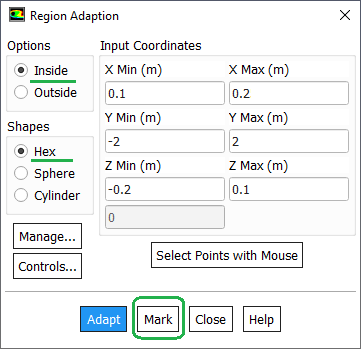#### Volumetric Heat Sources

ANSYS FLUENT expects heat sources in terms of heat density that is [W/m3] whereas STAR-CCM+ can take directly the heat generation rate [W] for a given volume. In order to check that the total applied heat generated rate is equal to the expected value, initialize the flow field and then calculate heat transfer rate from "Reports -> Fluxes" option. ANSYS FLUENT shall print the total "User Source" that is the total heat generation rate defined by all defined volumetric heat sources.

A sample SCHEME script to apply volumetric heat source of 500 [W/m3] to a zone named htr-ss and material defined as steel is as follows: define b-c solid htr-ss yes steel y 1 y 500 n no n 0 n 0 n 0 n 0 n 0 n 0 n no n. The energy should be turned ON and material name should be present before this script is run in TUI. This script is not applicable for rotating reference frames.

#### Convergence History

The residuals of continuity, momentum (u, v and w), energy (T), k and ε is used to check the convergence of iterative solution process. Convergence refers to the situation where the calculated value is assumed to tend to correct inversion of equation [A]{x}={b}.

Sometimes k (designated as Tke in STAR CCM+) and and ε (referred to as Tdr in STAR CCM+) may be as high as few thousand and the results are reasonable. The scale can be reduced by turning off the normalization for k and ε. Normalization takes the very first iteration's values to normalize all subsequent residuals. When solution already start with a good solution or guess value for k and ε, the first value is already very low, and residuals may not reduce further. It then sometimes can happen that the residual increases instead of decreasing even though the absolute (without normalization) value might still be very low.

In ANSYS FLUENT, the denominator to calculate the scaled residual for the continuity equation of a pressure-based solver is the largest absolute value of the continuity residual in the first 5 iterations. When iteration starts with a good solution, the largest residual of first 5 iterations may be low. Hence in subsequent iterations, significant reduction in order of magnitude of the residuals may not be observed even though the convergence can be deemed to be of good accuracy. Note 'scaling' and 'normalization' of residuals are different and interpretation should be different as well.

Under Relaxation Factors (URF): As per ANSYS documentation and training material, Pressure and Momentum URFs MUST sum to 1.00 and flow stability is best with smaller pressure URF (0.03 is not uncommon).

#### Thin Walls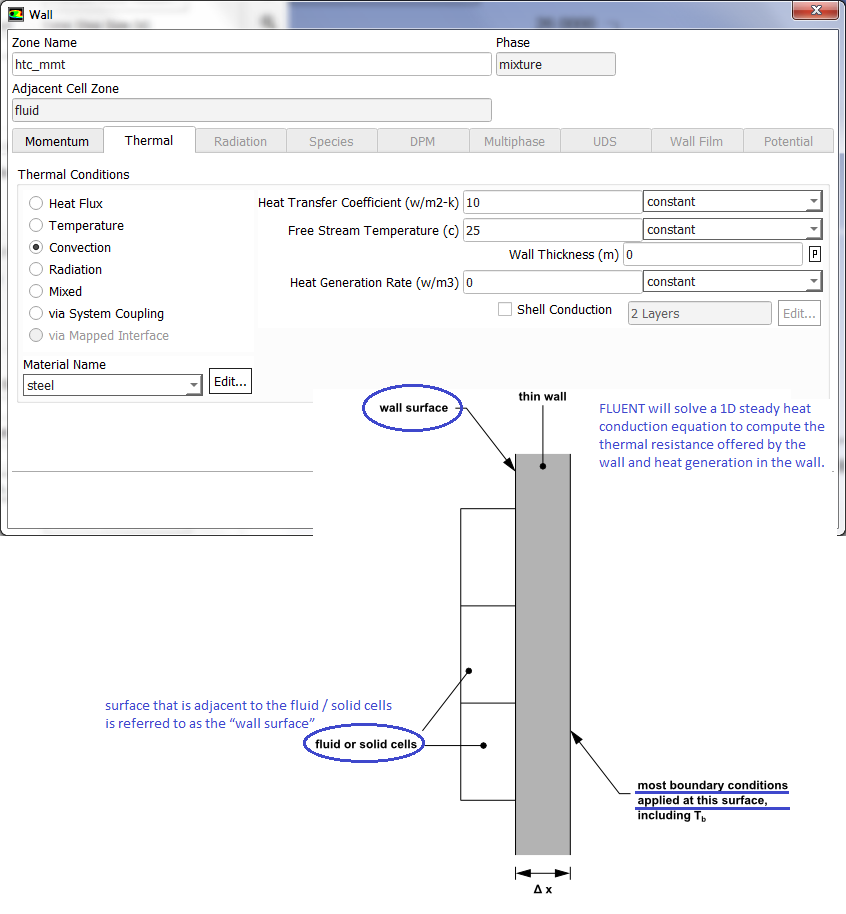Natural Convection (Buoyancy-driven Flows)

Boussinesq approximation: ρEFF = 1 - β(TWALL - TREF). Here: ρEFF= effective driving density β = coefficient of thermal expanison (equal to 1/T for ideal gas, T in [K]). Boussinesq approximation is only valid for β × (TWALL - TREF) << 1.0 say β × (TWALL - TREF) < 0.10. In other words, the temperature difference should be < 30 [K] for reference temperature of 300 [K].
1. Use Boussinesq approximation to start the simulation. Specify correct density and expansion factor of the fluid
2. Initialize the domain with small velocity say 0.02 or 0.05 [m/s] in the direction opposite to the gravity
3. Alternatively, solve a force convection flow with small velocity is expected inlet(s) and then switch on to natural convection mode
4. Turn-on radiation once flow and temperature fields have stabilized
When the Boussinesq approximation is not used, in buoyancy-driven flows, the body force [Fz = (ρ - ρOP)×g] acts in the momentum equation. Here, ρOP is operating density, default method to compute the operating density in ANSYS FLUENT is by averaging over all cells.As per FLUENT User's Manual: "The boundary pressures that you input at pressure inlet and outlet boundaries are the redefined pressures as given by equation above. In general you should enter equal pressures, p'HS at the inlet and exit boundaries of your ANSYS FLUENT model if there are no externally-imposed pressure gradients. For example, if you are solving a natural-convection problem with a pressure boundary, it is important to understand that the pressure you are specifying is p'HS. Therefore, you should explicitly specify the operating density rather than use the computed average. The specified value should, however, be representative of the average value. In some cases the specification of an operating density will improve convergence behavior, rather than the actual results. For such cases use the approximate bulk density value as the operating density and be sure that the value you choose is appropriate for the characteristic temperature in the domain."

#### Mesh Reordering - Node Renumbering

Both elements and nodes are numbered where elements are described as a set of nodes forming its vertices. The more compact is the arrangement of elements and nodes, lesser will be the memory requirements. Some terms associated with elements and node arrangements in a mesh are as follows.

• Bandwidth: It is the maximum difference between neighboring cells in a zone i.e. if each cell in the zone is numbered in increasing order sequentially, bandwidth is the maximum differences between these indices.
• Excerpt from user manual - FLUENT: Since most of the computational loops are over faces, you would like the two cells in memory cache at the same time to reduce cache and/or disk swapping i.e. you want the cells near each other in memory to reduce the cost of memory access.
• In general, the faces and cells are reordered so that neighboring cells are near each other in the zone and in memory resulting in a more diagonal matrix that is non-zero elements are closer to diagonal. Refer to "banded-matrices" in context with numerical simulations.

#### Solver Setting for Multi-Phase Flows

Multi-phase flows have wide applications in process, automotive, power generation and metal industries including phenomena like mixing, particle-laden flows, CSTR - Contunuously Stirred Tanks Reactor, Water Gas Shift Reaction (WGSR), fluidized bed, fuel injection in engines, bubble columns, mixer vessels, Lagrangian Particle Tracking (LPT). Some of the general characteristics and categories of multi-phase flow are described below along with setting parameters in ANSYS FLUENT

Multiphase flow regimes are typically grouped into five categories: gas-liquid (which are naturally immiscible) flows and (immiscible) liquid-liquid flows, gas-solid flows, liquid-solid flows, three or more phase flows. As can be seen, the immiscibility is a important criteria. In a multi-phase flow, one of the phase is usually continuous and the other phase(s) are dispersed in it. The adjective "Lagrangian" indicates that it relates to the phenomena of tracking a moving points ("fluid particles") - such as tracking a moving vehicle on a road. On the other hand, the adjective "Eulerian" is used to describe correlations between two fixed points in a fixed frame of reference - such as counting the type of vehicles and their speeds while passing through a fixed point on the road.

Gas-liquid flows are further grouped into many categories depending upon the distribution and shape of gas parcels. Three such types are described below.

Bubbly Flow: it represents a flow of discrete gaseous or fluid bubbles in a continuous phase.Slug Flow: This is characterized by flow of large gas bubbles in liquid.Annular Flow: Here one of the phase if confined to area near the wall forming an annular section.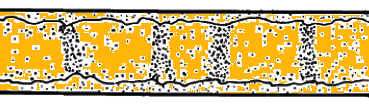Some other types of flows are particle-laden flow such as air carrying dust particles, slurry flow where particles are transported in a liquid, hydrotransport which describes densely-distributed solid particles in a continuous liquid such as cement concrete mix. Gas assisted mixing of solid such as fluidized-bed and settling tank where particles tend to sediment near the bottom of the tank forming thick sludge are some other examples of multi-phase flows.

The two dominant method of multi-phase simulations are listed below.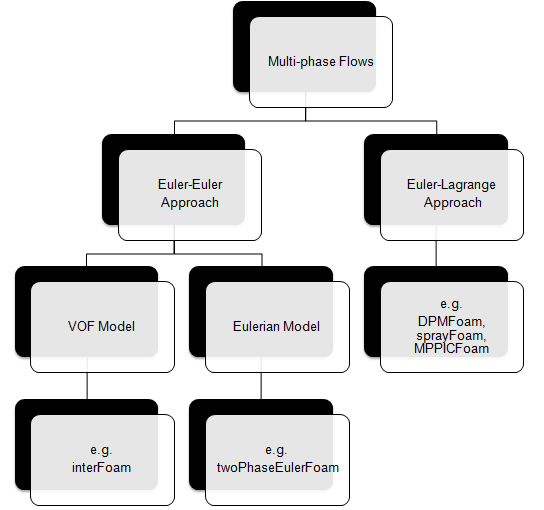In the Euler-Euler approach, the different phases are treated mathematically as interpenetrating continua where each phase coexist as continuous function in space and time.
• A phase cannot be occupied by the other phases in space, the concept of "phasic volume fraction" is used and assumed to be continuous functions of space and time. Distribution of phases are plotted by volume fraction whereas in DPM (Euler-Lagrange approach) the distribution of phases are plotted by particle track.
• Globally, the sum of this phasic volume fraction equals to one.
• Conservation equations for each phase are derived as a set of equations having similar structure for all phases, analogous of momentum equations.
• These equations are closed by constitutive relations obtained from empirical information.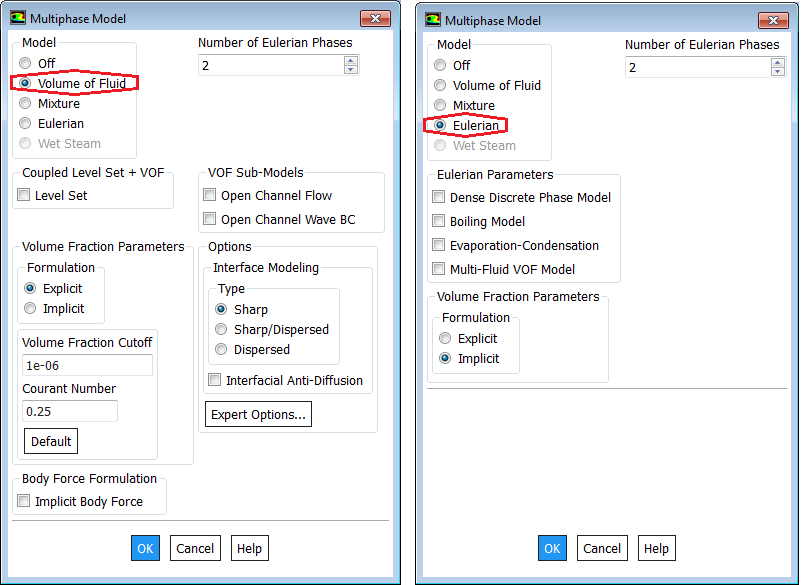In transient VOF simulations, ANSYS FLUENT solves the volume fraction equation(s) once for each time step (outer loop). To solve the volume fraction equation(s) at every (inner) iteration within a time step, use TUI: /define/models/multiphase/volume-fraction-parameters [solve vof every iteration?] yes. This will update convective flux coefficients in the other transport equations based on the updated volume fractions at each iteration, though this a computationally more expensive and less stable option. While using sliding meshes or dynamic meshes with layering and/or remeshing, using the solve vof every iteration? option will yield more accurate results, although at a greater computational cost.##### Atomization and Sprays

This formation of droplets from a liquid jet is known as Atomization. One of the dimensionless parameters that governs the formation of droplet is Weber Number. We = [ρL] × u2 × λ / σ where λ is radial integral length scale (d/8) and σ is the surface tension between liquid and surrounding gas. For single-phase nozzles and primary breakup distribution of droplets, estimate for the Sauter mean diameter of droplets as per Wu et al. (1992): d32 = 133 × We−0.74.

The Sauter mean diameter (SMD) is the diameter of a sphere that has the same volume to surface area ratio as the total volume of all the drops to the total surface area of all the drops. As per ANSYS (2016) Theory Guide, the most probable droplet diameter is d0 = 1.27 × d32 (1 − 1/n)1/n where n is the spread parameter (n = 3.5) for a single-phase nozzle.

##### Particle Depositions

particles tend to deposit on solar panels, turbine blades and even rotating blades of ceiling and exhaust fans in our houses. They gradually grow in thickness and density and remain stuck the blades till they are mechanially wiped away. This deposition predictions is computed in terms of sticking efficiency which tend to be high at lower diameter and low at higher diameters of the particles. The sticking efficiency is also dependent on particle Young's modulus and temperature of the surface where deposition is likely to occur. Most commercial programs such as ANSYS FLUENT has built-in boundary conditions to define the phenomena when particle strikes a boundary face. However, none of these boundary conditions accurately represent the particle-wall interaction which governs the rate of deposition and the deposition phenomena itself.

1. Reflect – elastic or inelastic collision
2. Trap – particle is trapped at the wall
3. Escape – particle escapes through the boundary
4. Wall-jet – particle spray acts as a jet with high Weber number and no liquid film
5. Wall-film – stick, rebound, spread and flash based on impact energy and wall temperature
6. Interior – particle passes through an internal boundary zone

The collision phenomena is defined in terms of co-efficient of restitution which is the ratio of the particle rebound velocity to the particle normal velocity. As the particle normal velocity decreases, the particle rebound velocity decreases and eventually reaches a point where no rebound occurs and the particle is captured. This velocity at which capture of a particle occurs is known as the capture/critical velocity. Brach and Dunn (59) formulated an expression to calculate the capture velocity of a particle using a semi-empirical model. In this model, the capture velocity of the particle was calculated based on the experimental data and is given as follows: VCRIT = (2E/DP)1.428 where E is the composite Young's modulus which is determined based on the Young's modulus of the particle and that of the wall.

##### De-fogging and De-icing
Condensation is a phenomenon that occurs when the headlamp is submitted to cold and moist environments. This will sometimes lead to fogging of the headlamps outer lens. ANSYS provides a tutorial on Windshield De-fogging Analysis using EWF Model where EWF = Eulerian Wall Film. Also, it is reported in "CFD Modelling of Headlamp Condensation" - Master’s Thesis in Automotive Engineering by Johan BrunbeRG and Mikael Aspelin, De-Fogging Module (DFM) was at first developed for de-fogging and de-icing simulations for HVAC applications. The DFM is written as a User-defined Function (UDF) and provided by ANSYS. Some assumptions and simplifications are made in the DFM and stated below:
1. Condensation will only occur at specified surfaces
2. The condensation film is fully contained by the first layer of cells adjacent to the specified walls
3. Condensation mass transfer rates are determined only by the gradient of water vapour mass fraction in the cells containing the condensation film
4. The condensation film is stationary
5. The inlet relative humidity is constant in time
6. Gravity and surface tension effects of the condensation film is neglected
7. The Diffusion coefficient of water vapour in moist air is known as a function of local pressure and temperature, Mollier chart or the Bahrenburg approach
8. Inlet and outlet mass flow rates

##### Discrete Phase Model: DPM
In Lagrangian particle tracking (Euler-Lagrangian approach), the discrete or dispered phase comprise of particles and bubbles. While the term 'particle' is used to represent the dispersed phase, it inherently refers to a 'parcle' or 'agglomeration' or 'assemblage' of similar particles. Note: in ANSYS FLUENT, in steady-state discrete phase modeling, particles do not interact with each other and are tracked one at a time in the domain.

The primary inputs that a user must provide for DPM caculations are the initial conditions that define the starting positions, velocities, parameters for each particle stream and the physical effects acting on the particle streams. Initial conditions of a particle/droplet stream is specfied by creating an 'injection' and by specifying its attributes. The initial conditions describe the instantaneous conditions of an individual particle which are:

• position (x, y, z coordinates) of the particles or parcels
• velocities (u, v, w) of the particle. For cone injections, velocity magnitudes and spray cone angle are used to define the initial velocities
• diameters of the particle
• temperature of the particle
• mass flow rate of the particle stream that will follow the trajectory of the individual particle/droplet though it is required only for coupled calculations
• additional parameters for special injections such as atomizer models
By default, the spread parameter for the Rosin Rammler distribution is 3.50 for all the atomizer models. To alter this spread parameter through the Text User Interface, execute command (rpsetvar 'dpm/atomizer-spread-param 3.75).If the field "Number Of Continuous Phase Iterations Per DPM Iteration" field is set to 0, FLUENT will not perform any discrete phase iterations. Excerpts from ANSYS FLUENT: rule of thumb to follow when setting the parameters above is that if you want the particles to advance through a domain consisting of N mesh cells into the main flow direction, the Step Length Factor × N ≈ Max. Number of Steps.

The particle positions are always computed when particles enter/leave a cell even if a very large length scale is specified. The time step used for integration will be such that the cell is traversed in one step.

Also, if "Number Of Continuous Phase Iterations Per DPM Iteration" field is reduced, the coupling between dispersed and continuous flow increases and vice-versa. For a closer coupling, this value should be set to a value ≤ 5 though no unique upper limit exists. Increasing the under-relaxation factor (setting value closer to 1) for discrete phase improves coupling and reducing the under-relaxation factor (setting value away from 1 and closer to 0) for discrete phase weakens the coupling.

DPM Trajectory Equation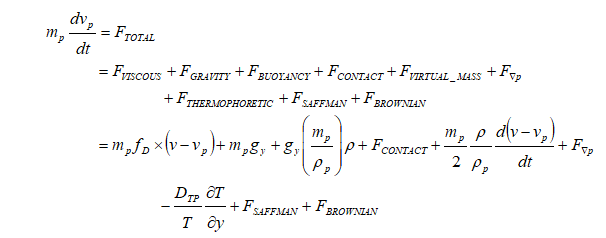Here subscript 'p' denotes 'particle'. Various empirical correlations of drag laws for viscous forces over solid particles are: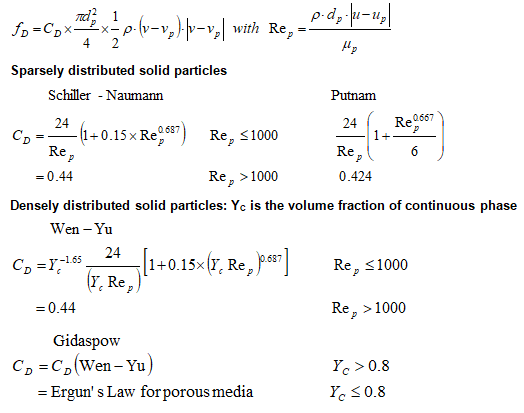ANSYS FLUENT calculates drag coefficient of non-spherical particle using shape factor defined as [s/S] where 's' is the surface area of a hypothetical sphere having the same volume as the particle and 'S' is the actual surface area of the particle.

As explained above, the underlying physics of DPM is described by ordinary differential equations (ODE) as opposed to the Navier-Stokes equation for continuous flow which is expressed in the form of partial differential equations (PDE). Therefore, DPM can have its own numerical mechanisms and discretization schemes, which can be completely different from other numerics (such as discretization schemes) to solve the governing equations. The Numerics tab gives users control over the numerical schemes for particle tracking as well as solutions of heat and mass equations related to particles - continuous phase interactions.Type of coupling: continuous (carrier) phase and discrete phase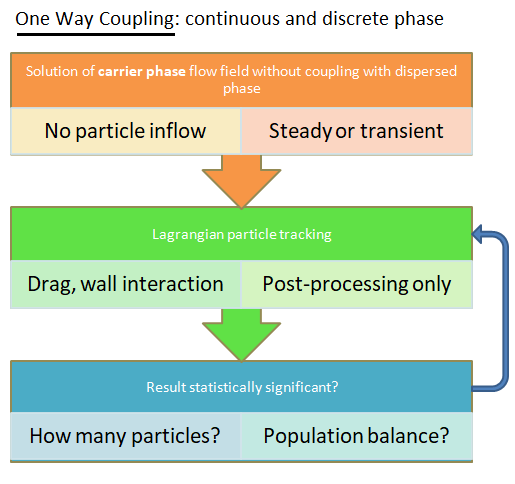The approach differs in ways injected particles (number, volume fraction, injection speed) are treated or the way particle-particle collisions are treated. For example: one particle can represent either one or a group of particles (sometimes known as parcels). In Discrete Particle Method (DPM), particle particle interaction can be treated in following 3-ways:
• no collisions at all (used for dilute suspensions): one-way or two-way coupling, Newton's equation of motion for dispersed phase.
• 3-way coupling - soft-sphere approach (the discrete element method, DEM): the instantaneous inter-particle contact forces due to oblique collisions (the normal, damping and sliding forces) are computed using equivalent mechanical elements springs, dashpots and sliders respectively. This approach requires a very small time step which can be as low as < 1 [μs].
• 3-way coupling - hard-sphere approach: interactions between particles are pair-wise (binary collision), instantaneous, momentum-conserving. Faster than soft-sphere method due to feasibility to use longer time steps.
For situations where volume concentration of discrete phase is high (> 10%), DDPM or Dense Discrete Phase Model or Dense DPM (described in ANSYS FLUENT) has been developed which is based on statistical treatment of the particle-particle interactions and can be classified as CPFD (Computational Particle Fluid Dynamics). [Reference: "The dense discrete phase model for simulation of bubbling fluidized beds: Validation and verification" by Schalk Cloete and Shahriar Amini] The DDPM is a Lagrangian parcel-based approach which models particle collisions and uncorrelated translations using the kinetic theory of granular flows. This approach results in better resolution of particle clusters, more natural incorporation of particle size distributions and better handling of crossing particle clusters.In ANSYS FLUENT, the DDPM option is available only under Eulerian multi-phase model.In two-way coupling, dispersed phase modifies carrier phase turbulence where bigger particles usually enhance turbulence and smaller particles damp turbulence. This leads to situation where carrier phase turbulence becomes anisotropic. On the other hand, carrier phase turbulence enhances dispersion (fluctuating flow field) and clustering of the dispersed phase (stagnant flow field). When the flow is turbulent, it imparts a random motion on the particles based in instantaneous components of fluctuating velocity. However, RANS flow solution does not resolve all the small–scale turbulent eddies in the flow. The effect of eddies on particle trajectories is resolved using stochastic tracking. Here, a number as specified by "number of tries", particle streams are released from the same point. Each one is given a random "kick" in each grid cell based on the calculated turbulent intensity. This will indicate how turbulence will modify the trajectories.

Type of injection

For typical applications, the computational cost of tracking all of the particles individually is prohibitive. Instead, the approach adopted in DPM and Discrete Element Method (DEM) Collision Model is that like particles are divided into parcels and then the position of each parcel is determined by tracking a single representative particle. Thus, in such calculations, following differences between DPM and DEM are mentioned in FLUENT user manual.
• The mass used in the DEM calculations of the collisions is that of the entire parcel, not just that of the single representative particle.
• The radius of the DEM parcel is that of a sphere whose volume is the mass of the entire parcel divided by the particle density.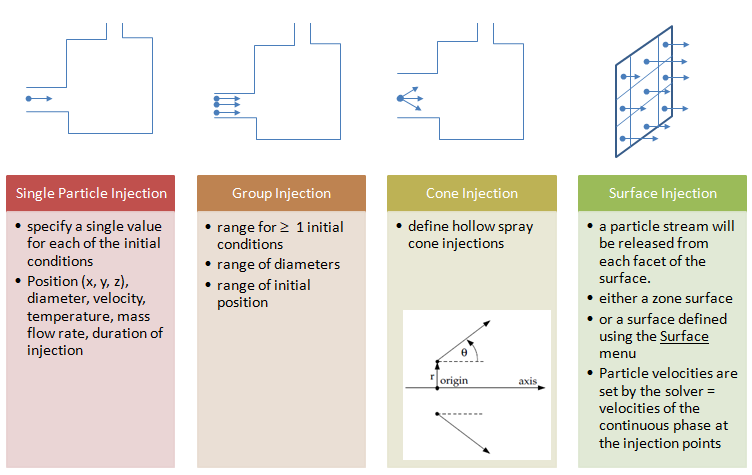In ANSYS FLUENT, for surface injection, mass flow rates of the particles from each face (each element of the boundary zone) can be scaled by the ratio of the area of the face particles are released from to the total area of the surface using option "Scale Flow Rate By Face Area" under Point Properties. Note that surfaces invariably have non-uniform distributions of points. In order to generate a uniform spatial distribution of particle streams released from a surface in 3D, one will need to create a bounded plane surface with a uniform distribution using the Plane Surface panel. However, this is applicable to rectangular surfaces only as the 'bounded' plane method does not create a circular surface.

Group injections requires to set the properties position, velocity, diameter, temperature and and flow rate for the First Point (φ1) and Last Point (φN) in the group. ANSYS FLUENT assigns a value of φi to the i-th injection in the group using a linear variation between the first and last values for φ. The specified flow rate is defined per particle stream and can also be interpolated linearly between mass flow rates of the first and last injection points.

In ANSYS FLUENT, particle initial conditions (position, velocity, diameter, temperature and mass flow rate) can also be read from an external file to describe injection distribution. The file has the following form with all of the parameters in SI units. All the parentheses are required, name is optional.

(( x y z u v w diameter temperature mass-flow) name)

Sample files generated during sampling of trajectories (path Reports → Sample → Set-up...) for steady particles can also be used as injection files since they have a similar file format. Note that one line in the file = one parcel, 10 lines in the file = 10 parcels, 100 lines in the file = 100 parcels ... "parcels" = groups of particles which have similar properties (diameter, velocity, temperature) as each particle. Parcels are used as computationally it is impossible to track very large number say millions of particles. The mass-flow of the parcel = mass-flow of the group of particles. Generally the more parcels you use, the more accurate the solution shall be.

Mass flow rate: Only for coupled calculations (discrete phase affecting flow field of continuous phase), the mass of particles per unit time that follows the trajectory can be defined in the injection setting option. Also note that the number of streams (say 100) is not the same as the number of particles. The DPM uses a parcel method where many particles are tracked in each parcel and ANSYS FLUENT allocates the number of particles in each parcel based on the particle diameter, density and mass flow rate. Numper of particles in each parcel NP = mPS × Δt / mP where mPS = mass flow rate in particle stream, mP = mass of each particle and Δt is the time step. Under steady state operations, it is assumed that any particle released from the same location with the same conditions will follow the same trajectory. P_FLOW_RATE macro can be used in a UDF to return the flow rate of a parcel in [kg/s].

Example: particle release from a surface - assuming there are 200 faces in the surface.

• The particle injected is sand with density of 2000 [kg/m3] and diameter 250 [μm]. Thus, mass of each particle is 8.18 [μg]
• Total mass flow rate of sand particles = 0.1 [kg/s].
• Total number of particles = 0.10 [kg/s] / 8.18×10-9 [kg] = 1.22×109 [particles/s]
• Thus, the 200 trajectories releases from 200 faces of the surface represents 1.22×109 actual [particles/s].
• If one defines a range of diameters by specifying "minimum diameter", "maximum diameter" and "number of diameters", the total number of trajectories computed will increase to 200 × "number of diameters".
• If stochastic tracking is switched on to account for Turbulent Dispersion and number of tries specified is 20, now the total number of trajectories computed will be 200 × "number of diameters" × "number of tries".

Number of Particle Streams: This option controls the number of droplet parcels that are introduced into the domain at every time step.

Note the options at top right quadrant of "Discrete Phase Model" setting window: Inject particles at (i)Particle Time Step and (ii)Fluid Flow Time Step. The option (i) requires two entrys (a)Particle Time Step Size in [s] and (b) Number of Time Steps. Option (ii) requires only (a) Particle Time Step Size. To start the particle injection at t = 0, set "Start Time" of inject = 0. In order to keep the particle injection long after the time period of interest, set a large value for the Stop Time, for example 500 [s] which ensures that the injection will essentially never stop.
Following calculator can be used to estimate the number of particles of specified diameter required to generate a known mass flow rate.
 Diameter of the droplets: DP [μm] Density of the particles: ρ [kg/m3] Mass flow rate [kg/s] Number of trajectories [-]

Poincaré plots are made by placing a dot on a given surface every time a particle trajectory hits or crosses that surface.

In ANSYS FLUENT, particles can be tracked and their status to be written to files when they encounter selected surfaces. For unsteady particle tracking, the particle trajectories will be updated and the particle states will be written to the files as they cross the selected planes or boundaries. Erosion and accretion rates can be accumulated for repeated trajectory calculations as described below.Summary information (number, mass, average diameter ...) for particles currently in the computational domain can be reported using the Particle Summary dialog box: Reports → Sample → Set-up... It will print following information (their application is mentioned along with the variable).

Total number of parcels, Total number of particles, Total mass, Maximum RMS distance from injector, Maximum particle diameter, Minimum particle diameter, Overall RR Spread Parameter, Maximum Error in RR fit, Overall RR diameter, Overall mean diameter (D10) - Comparisons / evaporation, Overall mean surface area (D20) - Absorption, Overall mean volume (D30) - Hydrology, Overall surface diameter (D21) - Adsorption, Overall volume diameter (D31) - Evaporation / molecular diffusion, Overall Sauter diameter (D32) - Combustion / mass transfer / efficiency studies and Overall De Brouckere diameter (D43) - Combustion equilibrium.

It is expected that a 'random' point generation method may yield a 'visually' uniform distribution of the points inside a disk. However, the probability function for distance 'r' from the center of a point picked at random in a unit disk is P(r)= 2.r and hence the point generated will be distributed as shown below.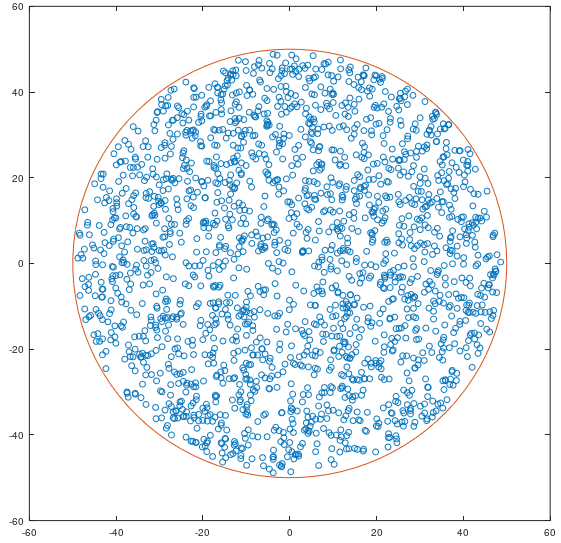The GNU OCTAVE script used to generate it is as follows.

 % Data - number of points, radius, centre of the circle n = 2000; RR = 50; xc = 0; yc = 0; % Random angular cocordinates q = rand(1, n) * (2*pi); r = rand(1, n) * RR * RR; x = xc + 0.98 * sqrt(r) .* cos(q); y = yc + 0.98 * sqrt(r) .* sin(q); a = linspace(0, 2*pi, 100); cx = RR * cos(a); cy = RR * sin(a); figure(); hold on; box on; plot(x, y, 'o');   % Plot the data points plot(cx, cy, '-'); % Plot the boundary hold off;
There is a Python code which can generate points iteratively to keep the points far enough to look uniform as shown below.A Python program to generate the relatively uniform distribution of points based on sprials originating from the centre of the disk is summarized below. Reference: stackoverflow.com/ questions/ 27606079/ method-to-uniformly-randomly-populate-a-disk-with-points-in-python

 import math import random import numpy as np import matplotlib.pyplot as plt n = 2000 R = 50 alpha = math.pi * (3 - math.sqrt(5)) #The "golden angle" phi = random.random() * 2 * math.pi x = np.empty((n,1)) y = np.empty((n,1)) for k in range(1, n): q = k * alpha + phi r = math.sqrt(float(k)/n) x[k-1] = 0.98 * R * r * math.cos(q) y[k-1] = 0.98 * R * r * math.sin(q) # for plotting circle line: a = np.linspace(0, 2*np.pi, 100) cx,cy = R * np.cos(a), R * np.sin(a) fg, ax = plt.subplots(1, 1) ax.plot(cx, cy,'-', alpha=0.5) #Draw circle boundary ax.plot(x, y, '.')     #Plot points ax.axis('square') plt.xlim(-R*1.05, R*1.05) plt.ylim(-R*1.05, R*1.05) plt.show()The recommended option is to independently mesh the injection plane and extract centroid of the triangles. This can be achieved by importing the vertices of the triangular elements and finding their centroid as arithmetic average of the coordinates.
##### Dense Discrete Phase ModelThe DDPM is based on concept of granular flows and compressible regime (that is, where the solids volume fraction is less than its maximum allowed value described in image above), a solids pressure (which is similar to thermodynamic pressure) is calculated independently and used for the pressure gradient term in the granular-phase momentum equation. The expression of solid pressure is

ps = [1+2(1 + e) α gr ]α ρp Θs

where e is the coefficient of restitution, gr is the radial distribution function - a correction factor that modifies the probability of collisions between grains when the solid granular phase becomes dense (may also be interpreted as the nondimensional distance between spheres), α is the volume fraction of solid, ρp is the density of solid particles and Θs is the granular temperature. The radial function tends to infinity as the volume fraction tends to the packing limit and it tends to 1 as the volume fraction approached zero (the dilute limit).

The term "granular temperature" has been borrowed from kinetic theory of gases and is a bit misnomer which does not represent any temperature in thermodynamic sense. It represents particle momentum exchange due to translation and collision leading to definition of kinetic and collisional viscosities respectively. The shear and bulk viscosities arising due to this phenomena is added to the solid stress tensor. The granular temperature for the solids phase is proportional to the kinetic energy of the particles’ random motion mathematically expression as Θs = 1/3[u'.u'] where u' is the fluctuating solids velocity in the Cartesian coordinate system. This is defined as an ensemble average of the particles’ random velocity within a finite volume and time period having particle number per unit volume as averaging basis.

The collisional part of shear viscosity:Kinetic viscosity as per Syamlal et al.Kinetic viscosity as per Gidaspow et al.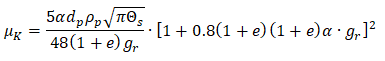The solids bulk viscosity accounts for the resistance of the granular particles to compression and expansion.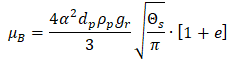##### Eulerian Wall Film
A wall film is a thin layer of liquid over a solid surface such as water dripping due to condensation. The surface tension is one of the dominant parameter which controls the thickness and flow rate of such liquid films. The Eulerian Wall Film (EWF) model can be used to predict the creation and flow of thin liquid films on the surface of walls. The thin film assumption is one usually made in both Eulerian and Lagrangian film modeling approaches, specifically that the thickness of the
• film is small compared to the radius of curvature of the surface so that the properties do not vary across the thickness of the film and
• films formed are thin enough so that the liquid flow in the film can be considered parallel to the wall, with an assumed quadratic shape.A thin liquid film forms as liquid drops impinge on a solid surface in the domain with several possible outcomes from this impingement:
• stick: where the droplet impacts the wall with little energy and remains nearly spherical
• rebound: where the drop leaves the surface relatively intact but with changed velocity
• spread: where the drop hits the wall with moderate energy and spreads out into the film and
• splash: where part of the impinging drop joins the film and another part of the drop leaves the wall in several other smaller drops.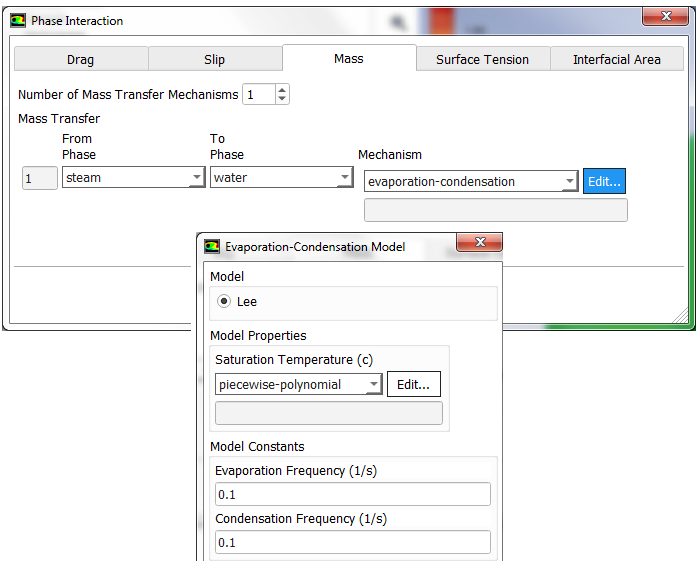##### Interface Tracking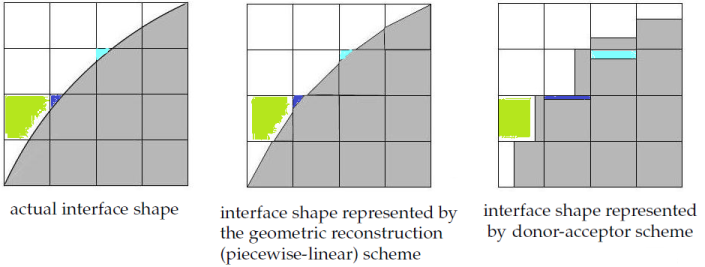The geometric reconstruction scheme represents the interface between fluids using a piecewise-linear approach. It assumes that the interface between two fluids has a linear slope within each cell and uses this linear shape for calculation of the advection of fluid through the cell faces.In ANSYS FLUENT this scheme has better accuracy as compared to other schemes (such as donor-acceptor scheme) and is applicable for general unstructured meshes.

The Algebraic Multi Grid solver is one among the family of multi-grid solvers. The adjective multi-grid refers to the concept that the solution process is implemented more than one grid (levels) even though the user has created only one grid.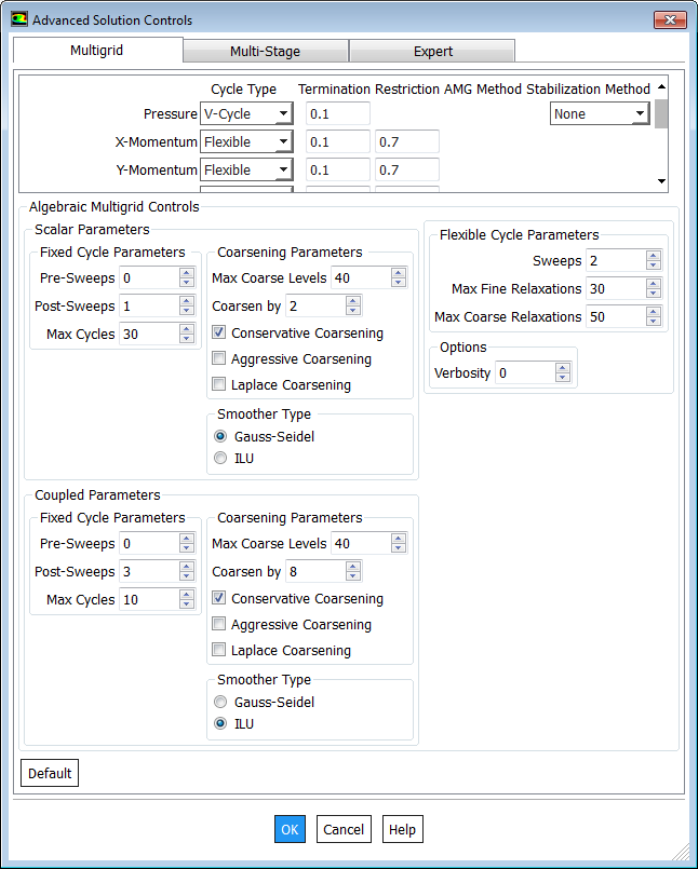In AMG solver, a 'virtual' coarse mesh equations are created by merging the original control volumes. The merged coarse control volume meshes are in general very irregular in shape. The coarse mesh equations thus impose conservation reduce the error components at longer wavelengths. The following image is taken from CFX user manual:Here, the results from coarse-mesh at each level is interpolated from/on the nearest finer mesh and vice-versa.

#### Transient Table as Boundary Condition in FLUENT

A time-history data such as inlet temperature as function of time or mass flow rate as function of time can be specified either as an UDF (cases where analytical equations can be derived) or as a data table.

The format of the standard transient profile file:

```(
(inletvel transdata 3 0)
(time  1 2 3 )
(invel 6 5 4 )
)```
Here '3' denotes the number of data points (number of rows in tabular format). '0' indicated that data is not time-periodic, use '1' for a time-periodic dataset. 'invel' shall appear as drop-down in boundary condition panel. Note:
• All quantities, including coordinate values, must be specified in SI units.
• Profile names (e.g. 'inletvel' in this example) must have all lowercase letters
• Data can be either in column or row format.

The data set used above can also be specified as described below:

```(
(inletvel transdata 3 0)
(time
1
2
3
)
(invel
6
5
4
)
)```

Tabular Transient Profiles

The format of the tabular transient profile file is as follows. Use TUI command "file read-transient-table fileTr.dat" to upload the data into the solver. The options "inletvel time" and "inletvel invel" shall appear in the drop-down menu of boundary condition panels.
```inletvel transdata 3 0
time   invel
1     6
2     5
3     4```

##### Interpolation of Results
Many a times we need to interpolate the results from previous simulations into a new simulation, such as when a new mesh is generated due to refinement or coarsening. Sometimes results may be available from simulations carried out in other software (such as FLUENT or OpenFOAM) and the field variables need to be read into a new software (STAR-CCM+). Most of the commercial program provide an options to import and export data from and into CGNS and/or CSV format. The data in this format can be used to exchange result data from one program to the other.Data Import into ANSYS FLUENT: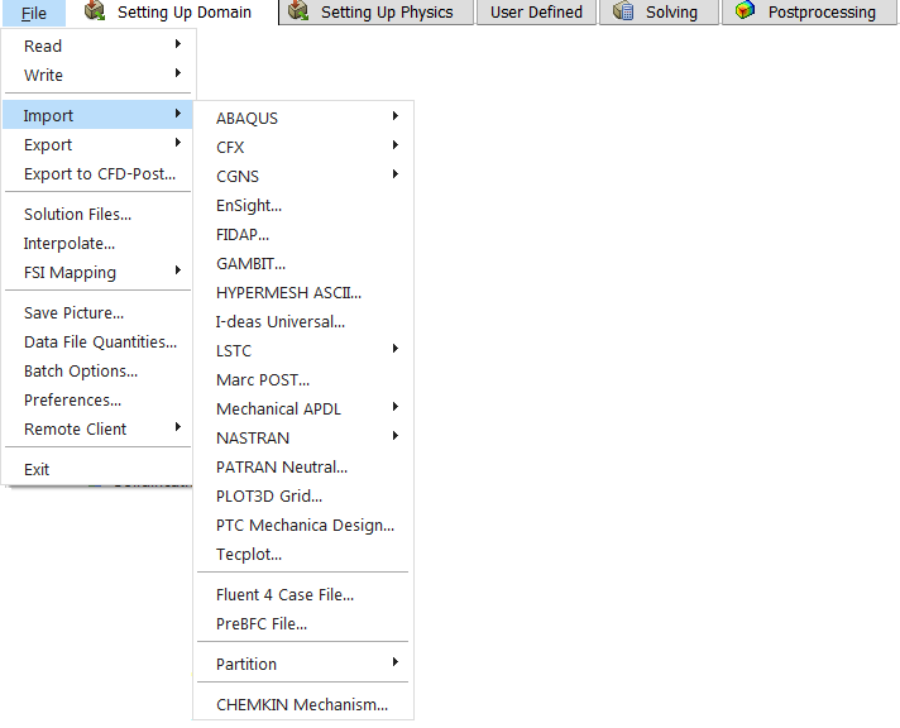Interpolation file format: ANSYS FLUENT

```2            --the interpolation file version
3            --dimension (2 or 3)
25000        --total number of points
3            --total number of fields (velocity, pressure...) included
x-velocity   --field variable to be interpolated
pressure     --field variable to be interpolated
-0.100000    --x-coordinate of first node in the list
-0.200000    --y-coordinate of first node in the list
-0.300000    --z-coordinate of first node in the list
...
...
...
1.000000    --x-coordinate of last node in the list
2.000000    --y-coordinate of last node in the list
3.000000    --z-coordinate of last node in the list
0.500000    --x-velocity at first node in the list
0.100000    --x-velocity at second node in the list
0.200000    --x-velocity at third node in the list
...
...
...
5.000000    --x-velocity at last node in the list
100.0000    --pressure at first node in the list
200.0000    --pressure at second node in the list
300.0000    --pressure at third node in the list
...
...
...
500.0000    --pressure at last node in the list```

At the end is a list of the field values at all the points in the same order as their names. The number of coordinate and field points (x-velocity and pressure in this case) should match the number given in line 3 (25000 in this case). Note that it cannot interpolate heat generation rate (W/m3), though internal energy, enthalphy, heat transfer coefficient... can be interpolated. In other words, no volumetric source/sink term can be interpolated in the fluid region. On the boundaries, all field variables are available for interpolation.

Data Export into CSV Format: ANSYS FLUENT:The header in CSV files for FLUENT and STAR-CCM+ uses different variable names. In STAR-CCM+ variables has to be specified with appropriate units: e.g. "Absolute Pressure (Pa)", "Velocity Magnitude (m/s)", "Velocity[i] (m/s)", "Velocity[j] (m/s)", "Velocity[k] (m/s)", "X (m)", "Y (m)", "Z (m)"... Note the space between variables and unit. The x-component of velocity is accessed by Velocity[i].

Data Mapper: STAR-CCM+: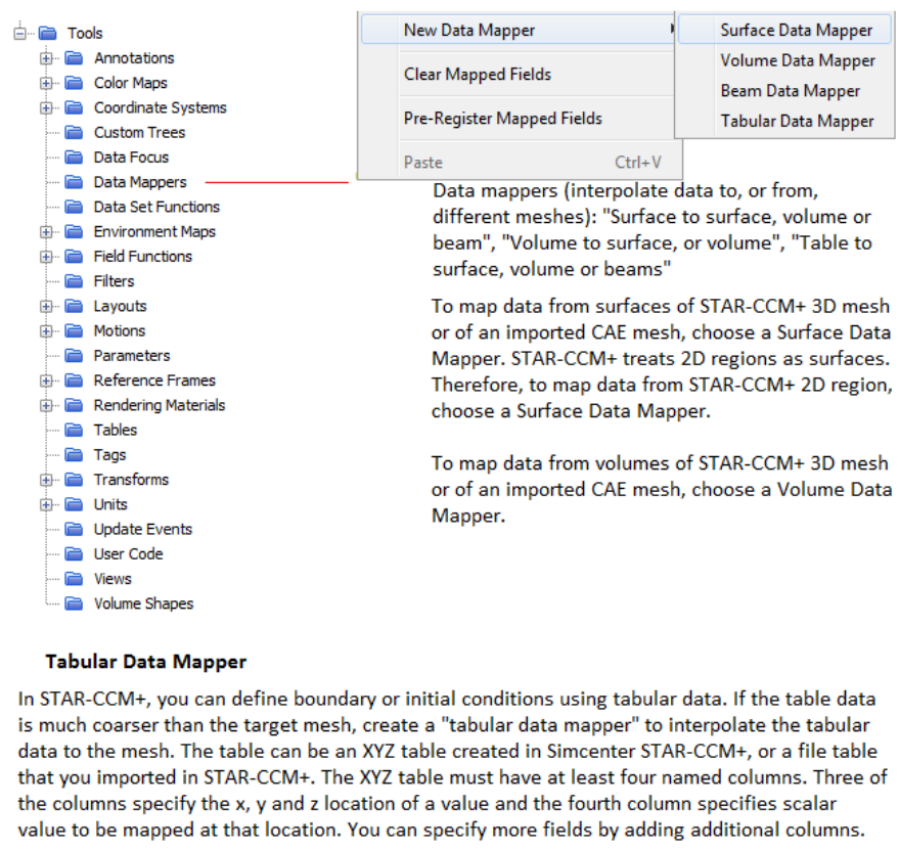Using a user field function, the table data which provides the variations of velocity with time can be imported and then defined as follow:

interpolateTable(@Table("VelData"), "Time", LINEAR, "VelMPS", \$Time)

Here the field function type should be vector. For boundaries such as inlet, in the “Physics Values” option of the boundary in the “Method” field, select Field Function, and then right below this, select the field function defined earlier.

The name of the table is VelData, Time is the name for the first column in the .csv file, LINEAR (other option available is SPLINE) is the interpolation method creating values between the discrete time values specified in the table, VelMPS is the name of the second column in the table (corresponding to the velocity magnitude), \$Time specifies that the velocity is function of time. In case velocity is function of spatial coordinates say y-axis: \$\${Position} can be used in place of \$Time to specify that the position coordinate is aligned with the y-axis (1 = y-direction, use '0' for x-direction and 2 for z-direction).

#### FSI: Fluid-Structure Interaction in ANSYS

FSI has wide application to check the effect of fluid forces on structural components such tank sloshing, flapping of wings and helicopter blades, pouring of liquid from a non-rigid container, pressure-limiting valves, leakage in fuel injectors operating at high pressure (such as Diesel engines) and check valves (deformable flow control devices) to name few.

FSI simulations are classified under a broader category of "Multi-physics Simulations" and not limited only to structural simulations. Fluid-thermal interaction, Fluid-thermal-electric interaction, Fluid-piezoelectric interaction, Structural-magentic interaction ... are other types of multi-physics simulations.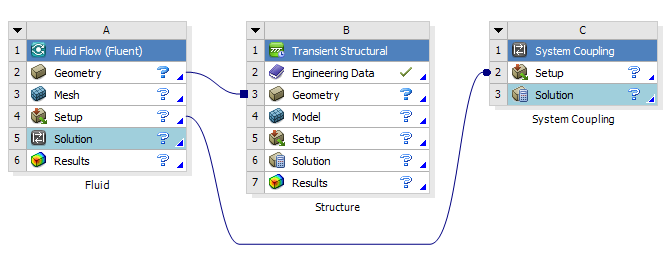In addition to the settings described above, following steps are required for a FSI simulation:
• Solution update can be done through System Coupling component only.
• System Coupling component overrides few settings in participant solver so that the time duration and time step settings are consistent across all participant solvers.
• The surface of solid boundary in contact with fluids need to be set as "Fluid-Solid Interface"in Transient/Static Structural Model.
• In fluid solver such as FLUENT, the "System Coupling" option needs to be activated under "Dynamic Mesh" tab.
• For FLUENT, all regions of type 'Wall' are shown in System Coupling setup.
• For (Transient/Static) Mechanical, all regions of type "Fluid-Solid Interface" appear in System Coupling component.
• Create solid-fluid coupling: select a fluid (FLUENT) and solid (Mechanical) region pair (use Ctrl key for multiple selection) and select "Create Data Transfer" from right click pop-up menu.
• "Data Transfer" defines the Source (participant solver, deforming region and variable being transfered e.g. force, displacement), Target (participant solver, deforming region and variable being transfered e.g. force, incremental displacement) and Data Transfer Controls (data transfer frequency, under-relaxation factor and convergence).
• Post-processing a FSI result:
1. Use 'Result' cell in Transient/Static Structural or Fluid Flow (FLUENT) for solver-specific post-processing
2. Connect structural 'Solution' cell directly to FLUENT system 'Results' cell or (c)Add a 'Results' System (ANSYS CFD-Post) for unified post-processing of structural and fluid results.

#### Parallel Processing

In order to reduce the simulation run time, parallel processing methods have been developed where the arithmetic involved with matrices are broken into segments and assigned to different processors. Some of the the terms associated with this technology are described below. The image from "Optimising the Parallelisation of OpenFOAM Simulations" by Shannon Keough outlines the layout of various components in a cluster.1. HPC: High Performance Computing - A generic term used to describe the infrastructure (both hardware and software) for parallel processing.
2. Cluster: A collection of workstations or nodes connected with each other with high speed network such as 1 GB/s Ethernet network or InfiniBand.
3. Node: Each independent component of a cluster or HPC set-up. Each node has following configurations:
• Chassis: For example - HP Z820
• CPU: For example - Intel XEON-E5 2687W
• GPU: Graphics Processing Unit e.g. NVIDIA
• Cores: For example - 8 cores per CPU @ 3.1GHz
• RAM: For example - 8GB DDR3-1600 Registered ECC memory
• Storage: For example - 1TB SATA HDD plus network file system (NFS) server
• Operating System: For example - CentOS 6.5
• Application Program: For example - CFD Software: OpenFOAM or FLUENT V17.2
• Latency: it refers to dormant capacity or sluggishness of the cluster interconnect. In other workds, it also refers to the inertia of the system. Lesser the latency, faster the response time.
4. Hyper-threading: It is a method which allows each core on the CPU to present itself to the operating system as two cores: one real and one virtual. The operating system can then assign jobs to the virtual cores and these jobs are run when the real core would otherwise be idle (such as during memory read/write) theoretically maximising the utilisation of the CPU.
• Excerpts from STAR-CCM+ User Manual: "Generally, for best performance, it is recommended that you turn off any features that artificially increase the number of cores on a processor, such as hyper-threading. In situations where hyper-threading is turned on to benefit other applications, you should generally avoid loading more processes than there are physical cores.
In addition to turning off hyper-threading, it is recommended that you set your BIOS settings to favor performance rather than power saving. Almost all heavily parallelized applications suffer performance problems if the CPU frequencies are spun up and down. In most parallel applications and STAR-CCM+ in particular, when one core is operating at a reduced frequency, all other cores are running at lower frequencies as well.
5. Memory Intensive Applications: This refers to the programs whose performance is more influenced by RAM than the clock-speed of the CPU. For example - OpenFOAM
6. Partitioninng: This is nothing but a "work distribution" among CPUs. However, tracking of nodes and elements are important. Excerpts from FLUENT user's manual: "Balancing the partitions (equalizing the number of cells) ensures that each processor has an equal load and that the partitions will be ready to communicate at about the same time. Since communication between partitions can be a relatively time-consuming process, minimizing the number of interfaces can reduce the time associated with this data interchange."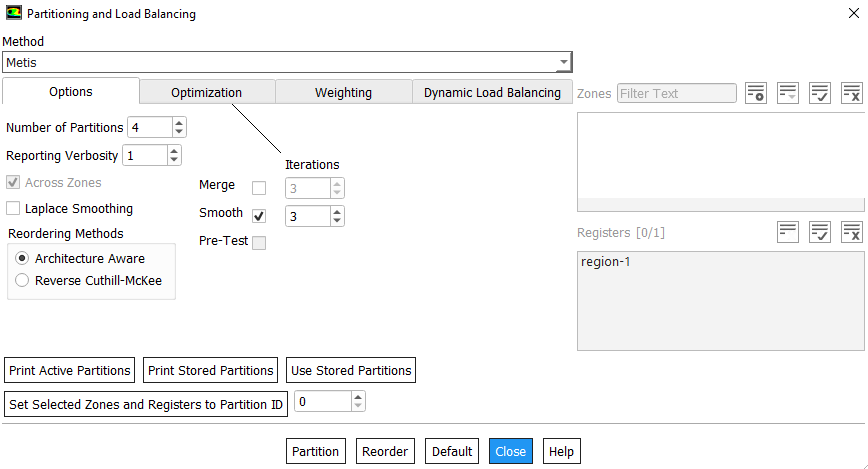7. MPI: Message Passing Interface - Each compute node is connected to every other compute node though the network and relies on inter-process communication to perform functions such as sending and receiving arrays, summations over all cells, assembling global matrix - remember A.x = b. Inter-process communication is managed by a message-passing library by an appropriate implementation of the Message Passing Interface (MPI) standard such as OpenMPI, Intel MPI, Cary MPI, IBM Platform MPI, Microsoft MPI, MPICH2 or vendor's own version of MPU.
8. Scalability: It refers to decrease in turnaround time for solutions as the number of compute nodes increases. However, beyond a certain point the ratio of network communication time (refer to definition of Cluster above) to computational time increases, leading to reduced parallel efficiency, so optimal system sizing is important for any simulations - determined from a ratio of the time to compute and the time that is taken to exchange data between cluster nodes.
9. Bandwidth of overall cluster interconnect: In ANSYS FLUENT, a table containing information about the amount of data communicated within one second between two nodes can be printed to the console. The table summarizes the minimum and maximum bandwidth between two nodes. Checking the bandwidth is particularly useful when you cannot see good scalability with relatively large cases.

In ANSYS FLUENT, one can reduce communication and improve parallel performance by increasing the report interval for residual printing/plotting or other solution monitoring reports. The most relevant quantity is the Total wall clock time which can be used to gauge the parallel performance (speedup and efficiency) by comparing this quantity to that from the serial analysis. Alternatively, an approximation of parallel speed-up may be found in the ratio of Total CPU time to Total wall clock time.

The comparison of two machines or clusters should be made on following parameters:

 Design Parameter Unit Value-1 Value-2 Remark CPU Operating Frequency [GHz] 3.5 4.2 Base clock speed, base clock frequeny Maximum Turbo Frequency [GHz] 4.5 4.8 Maximum Boost Clock Instructions per clock [M/cycle] 3.5 3.2 Parameter which decides actual computational speed RAM size per core [GB] 4.0 6.0 Cache Memory Size [MB] 32 16 Reduce time to access data from main memory Bus speed [GT/s] 8.0 6.0 Interconnect Speed [GB/s] 100 80 Such as infiniband Hyperthreading? [ - ] Yes No No qunatitative impact on computation speed
##### Specification of a Workstation
 Speed: 3.5 GHZ minimum Storage: 8 TB HDD: 3.5" 7200 RPM SATA Memory: 128 GB SO-DIMM DDR4 2400MHz Display Type: 24" LCD Optical Drive: Yes Speaker: with microphone Graphics: Integrated AMD Radeon Graphics or Quadro 580 Camera: Integrated Networking: Integrated 100/1000M Keyboard: Yes Pointing Device: USB Calliope Mouse, Black WiFi Wireless LAN Adapters: 11AC (2x2), Bluetooth 5.0 Rear Ports: 4xUSB 2.0, 1 x line-out (3.5mm), Ethernet (RJ-45), HDMI 1.4 Front Ports: 2xUSB 2.0, 2xUSB 3.2 Gen 1 Headphone / microphone: combo jack (3.5mm), 1xmicrophone (3.5mm) Software Preload: Windows 11 MS-Office 2020

##### Making FLUENT Runs on Clusters
FLUENT runs on a cluster can be started either in [A] interactive mode (as if it is running on your local machine with all functionality of a GUI) or [B] in a batch mode with GUI OFF and graphics ON or [C] batch mode with GUI OFF and graphics OFF. In case of mode 'C', the runs can only be controlled and monitored either through text files and/or through FLUENT Remote Visualization interface. Note that the HPC server needs a trigger from the solver to stop or exit the job when there is any error while iterating. As per FLUENT user guide: "Batch option settings are not saved with case files. They are meant to apply for the duration of the current ANSYS FLUENT session only. As batch options are not saved with case files, journal files developed for use in batch mode should begin by enabling the desired batch option settings". The TUI command that should be added to the journal file before iterate statement is /file/set-batch-options n y y n where n, y, y and n stands for answer to "Confirm Overwrite?", "Exit on Error", "Hide Questions" and "Redisplay the Questions" respectively.

Graphics refers to the pictures, videos, charts and animations. The graphics components of a computer control and enhance how these 'graphics' are displayed on computer screen. Usually the graphics components are on a separate card that is plugged into a slot on the motherboard though these can and are built directly into the motherboard as well. Some other names used for the graphics components are video card, video adapter and display adapter. NVIDIA Tesla GPUs for servers and workstations, Quadro GPUs for workstations and GeForce GPUs - gaming class cards are few examples of GPU.

Similar to an operating system which deals with how a computer starts and shuts down, the graphics driver is a program that enables computer to use the graphics card and communicate with CPU, HDD and other components. A 'driver' in general is a software component that lets the operating system and a 'device' communicate with each other. E.g. there is USB 'driver' to make a USB mouse work. The graphics card sends the signal to the computer display and makes the data visible.

Note that computer 'monitors' are not same as "graphics cards or graphics drivers". Graphics card is a hardware and graphics driver is software to make it work. AMD Radeon a graphics card used in my laptop manufactured by Lenovo.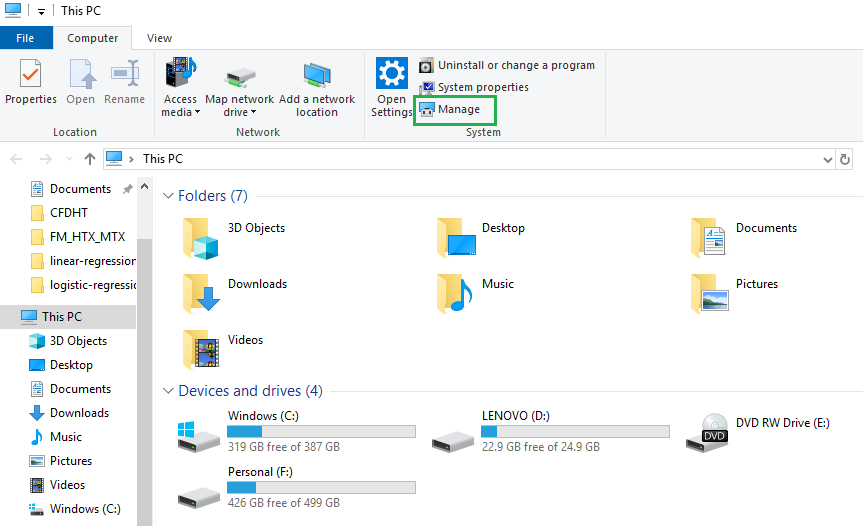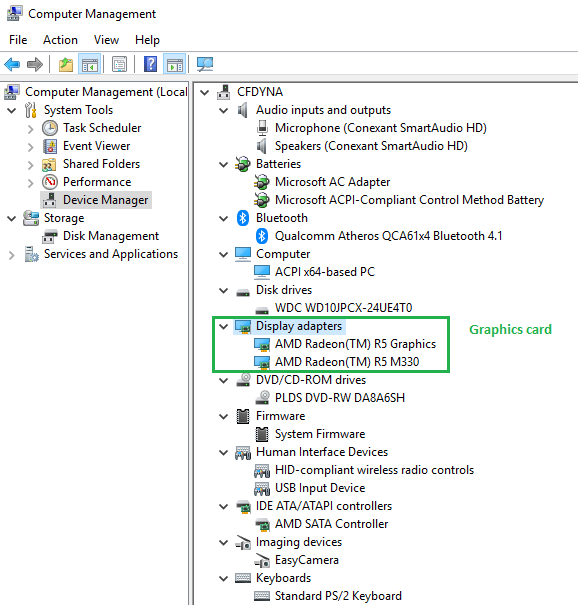Starting FLUENT in batch mode in Linux

 fluent 3d -g -i batch.jou -tN -driver opengl -rx 19.2 -mpi=hp -cnf=hosts.txt >& batch.out 2d -gr -t8 x11 19.1 intel 2ddp -gu -t32 null 19.0 ms 3ddp -t96 mpich2

Starting FLUENT in batch mode in Windows

 fluent 3d -g -i batch.jou -tN -driver opengl -rx 19.2 -mpi=hp -cnf=hosts.txt -wait 2d -gr -t8 x11 19.1 intel -hidden 2ddp -gu -t32 null 19.0 ms 3ddp -t96 mpich2
• -g indicates that the program (Cortex) is to be run without the GUI and graphics (Linux) or without graphics and with GUI minimized in the taskbar (Windows).
• -gr will run Cortex without graphics. This option can be used in conjunction with the -i option to run a job in background mode.
• -gu will run Cortex without the GUI but with the graphics window(s). (On Windows: it will run FLUENT keeping the window minimized that is the GUI will be available)
• -i reads the specified journal file say batch.jou
• -tN where N = number of processors = 2, 3, 4, ... 8, ... 32, ...
• -driver allows to specify the graphics driver to be used in the solver session
• -gu -driver null allow the generation and export of graphics files from FLUENT simulation run in batch mode
• hosts.txt is the file with the names of the computational nodes (or the hosts)
• Fluent 19.0 onwards, the solver does not differentiate between a serial mode and a parallel mode. Now the serial mode is in fact the general parallel mode with just one parallel task, -t1 option explained above.
• For a ANSYS FLUENT session that is already in progress, the graphics window display can be disabled using the TUI command: display → set → rendering-options → driver → null
• For a ANSYS FLUENT session that is already in progress, to re-enable a graphics window display that had been previously disabled, use the TUI command: (close-all-open-windows); display → set → rendering-options → driver → opengl

Reference: doku.lrz.de/pages/viewpage.action?pageId=27394531
``` WARNING: Rank:0 Machine mpp2-xxxxxxx has 37 % of RAM filled with file buffer caches.
This can cause potential performance issues. Please use -cflush flag to flush the cache.
(In case of any trouble with that, try the TUI/Scheme command '(flush-cache)'.) ```
In order to clear the buffer cache, add following lines at the beginning the journal file.
```(rpsetvar 'cache-flush/target/reduce-by-mb 12288)
(flush-cache)```
The number 12288 [Mb] = 12 x 1024 [kb] is the specification of the amount of system memory which cannot be flushed out due to boot image of Linux OS files in the memory of the diskless compute nodes. This means that on a compute node with 64 Gb, it tries to flush 64 [Gb] - 12.0 [Gb] = 52 [Gb] of memory. The value of 12288 also varies from cluster-to-cluster and may also change over the time.

The performance meter stores the wall clock time elapsed during a computation as well as message-passing statistics. Since the performance meter is always activated, the statistics can be printed after the computation is completed. To view the current statistics, use the Parallel/Timer/Usage menu item or TUI: paralle/timer/usage. There are many parallel statistics, few easy to decipher are:

• Average wall-clock time per iteration: it describes the average real (wall clock) time per iteration.
• Message count per iteration: it describes the number of messages sent between all processes per iteration. This is important with regard to communication latency especially on high-latency interconnnects. A higher message count indicates that the parallel performance may be more adversely affected if a high-latency interconnect is being used. Ethernet has a higher latency than Myrinet or Infiniband. Thus, a high message count will more adversely affect performance with Ethernet than with Infiniband.
• Data transfer per iteration: this factor describes the amount of data communicated between processors/iteration. This is important with respect to interconnect bandwidth and is usually dependent on the algorithm being used and the problem being solved. This number generally increases with increases in problem size, number of partitions and physics complexity.
• LE wall-clock time per iteration: the factor that describes the time (wall-clock) spent doing linear equation (LE) solvers (i.e. multigrid).
• Total wall-clock time: it describes the total wall-clock time which can be used to gauge the parallel performance (speedup and efficiency) by comparing this quantity to that from the serial analysis. An approximation of parallel speedup may be found in the ratio of Total CPU time to Total wall clock time.
In general, reduction in communication and improvement of parallel performance can be achieved by increasing the report interval for residual printing/plotting or other solution monitoring reports.
• The algebraic multigrid (AMG) solver for Fluent simulations is computationally intensive and in flow-only problems typically the coupled solver spends about 60~70% of run-time solving the linear system using AMG.
• On the other hand, segregated solver spends only 30~40% of run-time in AMG computation. One can find out AMG portion of a Fluent calculation (and therefore whether it is a good candidate for GPU employment) by adding the command '/parallel/timer/usage' to the journal file for a CPU-only run.
• The information is reported towards the end of the output file after successful completion of calculations. Output message would look something like: "LE wall-clock time per iteration: 5.678 sec (67.8%)".
• Additionally, stiff matrices are difficult to solve and require more inner-loop iterations in the AMG solver.
• Add these TUI in scheme file: parallel/latency and parallel/bandwidth.

Activating GPU in FLUENT runs

GPGPU stands for General Purpose GPU. The available GPU can be check in FLUENT TUI suing scheme command: /parallel/gpgpu. The GPGPU can be turned on for specific equation solver by TUI: /solve /set /amg-option /amg-gpgpu-option. The options are k, ε, temperature and pressure-velocity coupling.
##### Material Definition in Text File
Material properties in OpenFOAM is always defined in a text file. Similarly, FLUENT can read material in *.scm file as per following format. The extension 'scm' stands for SCHEME file.
```(
(air
fluid
(chemical-formula . #f)
(density (constant . 1.225)
(premixed-combustion 1.225 300))
(specific-heat (constant . 1006.43))
(thermal-conductivity (constant . 0.0242))
(viscosity (constant . 1.7894e-05)
(sutherland 1.7894e-05 273.11 110.56)
(power-law 1.7894e-05 273.11 0.666))
(molecular-weight (constant . 28.966))
)

(aluminum
solid
(chemical-formula . al)
(density (constant . 2719))
(specific-heat (constant . 871))
(thermal-conductivity (constant . 202.4))
(formation-entropy (constant . 164448.08))
)
)
```
When FLUENT opens, it auto executes the .fluent file in the: home directory of Linux users, C:\ directory of Windows machines. A .fluent file can contain any number of scheme file names to load. Unless full path is specified for each scheme file in .fluent file, FLUENT tries to locate the scheme files from the working directory. Sample .fluent file is described below. #t is to set boolean flags TRUE and #f is to set it FALSE.
```(load "plot.scm")
(lambda args
(apply old-rc args)
(if (cx-gui?)
(begin
;Do the customization here
(rpsetvar 'residuals/plot? #t)
(rpsetvar 'residuals/settings '(
(continuity #t 0 #f 0.00001)
(x-velocity #t 0 #f 0.00001)
(y-velocity #t 0 #f 0.00001)
(z-velocity #t 0 #f 0.00001)
(energy #t 0 #f 1e-06)
(k #t 0 #f 0.00001)
(epsilon #t 0 #f 0.00001)
)
)
(rpsetvar 'mom/relax 0.4)
(rpsetvar 'pressure/relax 0.5)
(rpsetvar 'realizable-epsilon? #t)
(cxsetvar 'vector/style "arrow")
)
)
)
)
)```

#### Troubleshooting Convergence in FLUENT

For all iterative solvers, the solution is quickly and smoothly achieved if the initial guess is closer to expected value. This is not always possible in CFD simulations with mean flow direction not aligned to coordinate system. The standard approach to handle such situation is as follows:

Step-01: Start with laminar or turbulent flows with firs order (Upwind) discretization schemes. Use incompressible fluid properties. Turn off energy equation - that is temperature field shall not be solved. Run for 500 iterations.

Step-02: While keeping incompressible fluid properties, turn ON energy equation - that is temperature field shall be solved. Run for 1000 iterations.

Step-03: While keeping incompressible fluid properties, energy equation ON, change discretization scheme for momentum to second order [Central Difference Scheme]. Run for 500 iterations.

Step-04:Change fluid properties to ideal gas or incompressible-ideal-gas as the case may be.

Step-05:Optionally, change the discretization scheme for turbulence parameters (k, ε ω) to second order.

All these steps can be build into a single journal script while making batch runs and there is not need to make separate runs for each step mentioned above.

Error message: Divergence detected in AMG solver: temperature

1. Reduce explict-relaxation in TUI:
• (rpsetvar 'temperature/explicit-relax? #f)
• (rpsetvar 'explicit-relaxation? #t)
• (rpsetvar 'temperature/explicit-relax 0.1) - By default, explicit-relax is set to 1.0.
2. Turn OFF secondary gradient on all the cells: (rpsetvar 'temperature/secondary-gradient? #f). By default, this is #t (true). To obtain the value, type: (rpgetvar 'temperature/secondary-gradient?) which will return either #t or #f.
3. Turn OFF secondary gradient in shell conduction zones: (rpsetvar 'temperature/shell-secondary-gradient? #f)

#### ANSYS Workbench

ANSYS has an option to mantain the geometry, mesh, solver and post-processing application inside a common project structure defined as Analysis Systems and Component Systems. Analysis system is a 'Set' of all necessary Component Systems while the later comprise of standalone editors to build the projects.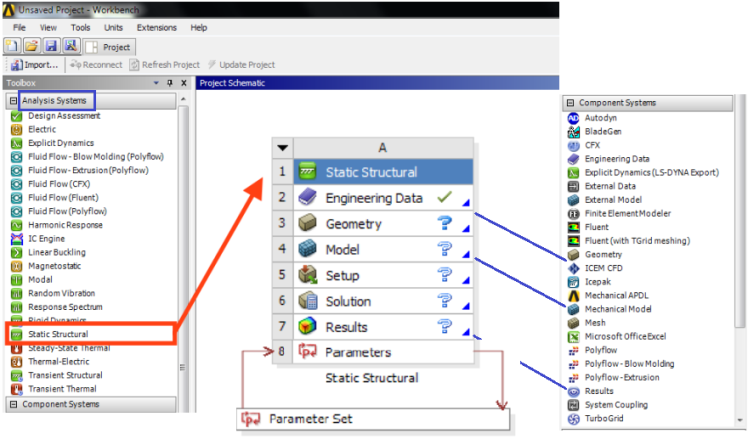In the diagram shown above, the individual constituent Component Systems of an Analysis System is described. Following is a demonstration of Static Structural and Fluid Flow (Fluent) Analysis System.In the project schematic shown below, the Analysis System is constructed from individual Component Systems with a parametric study defined for Geometry, Mesh and Boundary Conditions.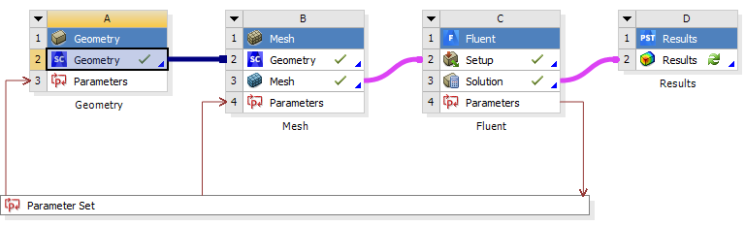##### Reduced Order Modeling
A Reduced Order Modeling (ROM) is one of the basic requirements of creating Digital-Twins. Following steps need to be followed to create a ROM in ANSYS FLUENT.

Step-01: Open "Fluid Flow (Fluent)" from Analysis Systems branch in Workbench. This will create a FLUENT simulation tree containing Geometry, Mesh, Solution and Results branches. You can also 'Import' a Case and Data file create out of Workbench. This will automatically delete Geometry and Mesh tabs (branches).

Step-02: Open FLUENT session and expand "Parameters & Customization" branch under Model Tree. Create required numbers of "Input Parameters" and "Output Parameters". Note that the Input Parameters are the variables defined under "Boundary Conditions" which automatically appear under "Parameters & Customization" branch. Create Output Parameters" either under Parameters & Customization tab or through "Report Definitions" by creating a customer report where there is a checkbox to "Create Output Parameters".

Step-03: Once you define any Parameter in FLUENT or CFD Post session, "Parameter Set" branch gets added to the project schematics. Open this tab and add Design Points under Input Parameters columns. Click "Update Design Points" to let FLUENT solver update the "Output Parameters" which are shown by lightning symbol.

Step-04: Define ROM in TUI: "define models addon 11" - this will make ROM appear in model tree. Turn-on ROM and define 'Variables' and 'Zones' to be monitored.

Step-05: Save and close the FLUENT session. Update the Workbench project. Use Workbench -> View -> Files to check ROM snapshot file. There is also a *.rommesh file which stands for mesh for ROM.

Step-06: From "Design Exploration" section, drag-and-drop "3D ROM" inside project schematics.

Step-07: Define "Design-of-Experiment" parameters such as "Design of Experiment Type", "Number of Samples" (to meed desired resolution...

Step-08: Specify ranges for each input parameter. Click on the parameter name and adjust "Lower Bound" and "Upper Bound" as needed.

Step-09: Preview parameter which shows DOE table (all the combination of Input Parameters and Output Parameters that shall be solved). The lightning symbol under columns of Output Parameters indicate that they are yet to be solved for.

Step-10: Update the DOE run table, analyse the results - both flow/thermal and DOE error norms.

#### Solver Settings in COMSOL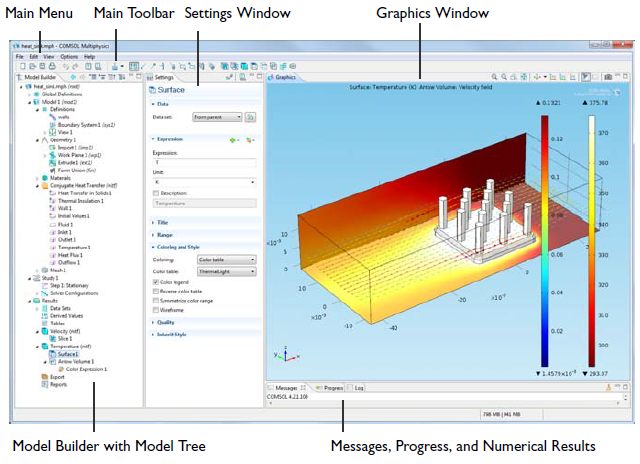A Solution node has an asterisk in the icon to indicate the sequences that have been edited.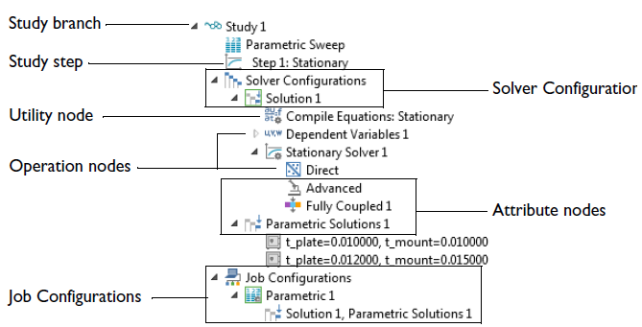The Non-Linear Solver Log: The log from the nonlinear solver contains the following information.
• The iteration number (Iter)
• A relative error estimate, which is one of the following:
• The solution error (SolEst), if Solution is selected from the Termination criterion list for the solver.
• The residual error (ResEst), if Residual is selected from the Termination criterion list for the solver.
• Both the solution error and the residual error (SolEst and ResEst), if Solution or residual or Solution and residual is selected from the Termination criterion list for the solver. The convergence is then based on the minimum of the solution error or the residual error multiplied by the residual factor.
• If the solver log detail is set to Detailed from the Solver log list in the Advanced node’s Settings window, the residual error and the solution error (depending on the selected termination criterion) from each individual field in the model appear in the solver log.
• The damping factor used in each Newton step (Damping).
• Fraction of Newton and Cauchy steps for the Double dogleg solver (Newton, Cauchy).
• The size of the undamped Newton step (Stepsize) in the error estimate norm.
• The numbers of residuals (#Res), Jacobians (#Jac), and linear-system solutions computed (#Sol) so far.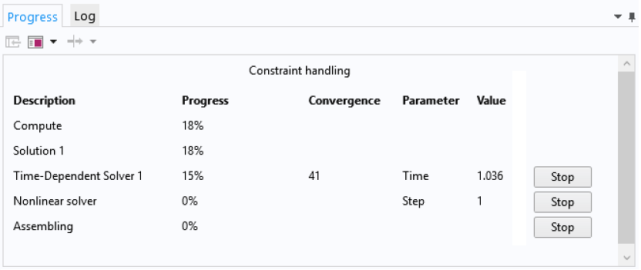The Progress window has the following columns:
• The Description column shows the solver name.
• The Progress column shows an estimate of the solver progress.
• The Convergence column shows an estimate of the solver’s convergence if available.
• The Parameter and Value columns contain solver-dependent information: the adaptive solver shows the adaptive mesh generation number, the time-dependent solver shows the time, the parametric solver shows the parameter value and the nonlinear solver and iterative linear system solvers show the iteration number.
The solvers call each other in a hierarchical order: the adaptive solver calls the linear, nonlinear, parametric, eigenvalue or time-dependent solver. The parametric solver calls the nonlinear or linear solver. The time-dependent, eigenvalue, linear and nonlinear solvers all call both the assembly and the linear system solver. The solver hierarchy is visible in the Progress window because each solver adds its own line when it is called.
##### CANCEL OR STOP A SOLVER PROCESS
You can cancel or stop the solver process if the solving time or likelihood for convergence is not progressing as expected. Use the horizontal or vertical scrollbars if needed. On the status bar you can follow the progress, cancel the solver process by clicking the Cancel button or click the Progress button to open this window. In the same way you can also cancel the meshing and the evaluation during postprocessing.

You can use the Stop buttons that appear at each solver level to stop the execution. When you click a Stop button, COMSOL returns a current approximation to the solution if possible. For example, when you click it at the adaptive solver level, the underlying linear, nonlinear, parametric, eigenvalue or time-dependent solver continues until it is finished, but the adaptive solver stops at its current generation, immediately returning a solution.

Similarly, you can click the Stop button to return the current iteration for the nonlinear solver or an iterative solver. Also use the Stop button during time stepping to return all time steps up to the current one. The parametric solver works similarly: to return the solutions for all parameter values up to the current one, click the Stop button.

##### Contacts and Interfaces
Excerpts from COMSOL Reference Manuals:

Pairs are available for assemblies (that is, geometries created by not forming a union of all geometry objects as the final step), where there is a need to connect boundaries between parts. By default, pairs are created automatically when forming an assembly. Contact pair modeling requires the Structural Mechanics Module or MEMS Module. There are two types of pairs - identity and contact.

###### IDENTITY PAIRS
An identity pair by default makes the fields across two connected boundaries (one from each connecting object in an assembly) continuous. This is equivalent to the continuity that is obtained by default on interior boundaries in a geometry created by forming a union. Some physics provide special boundary conditions for identity pairs to model slit conditions such as resistive layers. You can specify boundary conditions for these pairs from the Pairs submenu at the bottom of the boundary condition part of the context menu for the physics feature node.

In case you want to merge the pair created by slit operation, use Fuse Boundary Zone operation. It merges nodes, faces and works only on face zones. Note that Merge Zones does not cause any topological changes in the mesh - only new groupings of mesh are created (which is mostly irreversible). It is analogous to operation "Surface Grouping" in CFD-Post. The purpose is to reduce the number for zones for boundary condition settings and ease of selection of zones during post-processing operation.

###### CONTACT PAIRS
A contact pair defines boundaries where the parts can come into contact but cannot penetrate each other under deformation for modeling of structural contact and multiphysics contact.#### Workflow for AcuSolve

AcuConsole is the pre-post processor for solver AcuSolve which is based on the Galerkin/LeastSquares (GLS) finite element method. AcuFieldView is an OEM version of Intelligent Light’s FieldView CFD post-processor.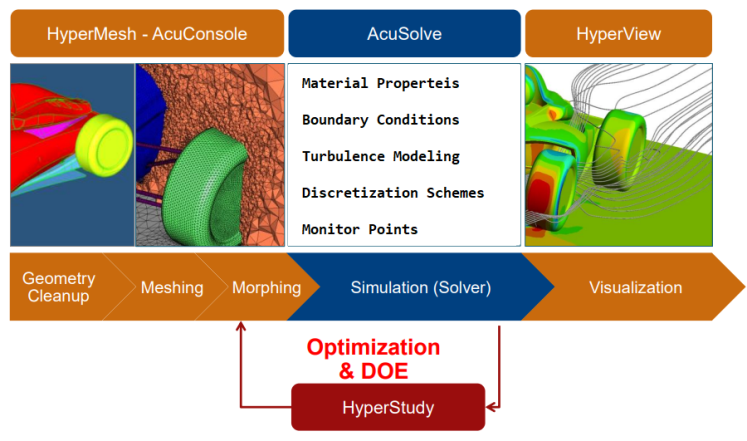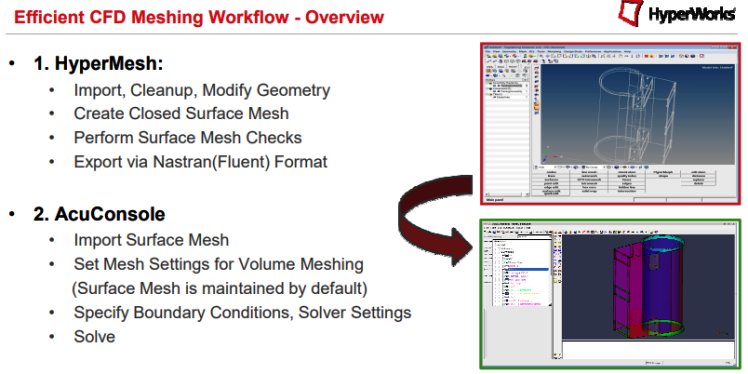#### Dimensionless Numbers

• CFL NUMBER: CFL, it is ratio of characteristic flow velocity to the speed with which time-marching solution advances over a mesh.
• Knudsen number: Kn, defined as the ratio of the mean-free path to the characteristic length scale.
• Mach number: M, defined as the ratio of the characteristic velocity to the sound speed
• Reynolds number: Re, defined as the ratio of the product of the characteristic velocity × characteristic length to the kinematic viscosity. This is one of the widely used number required to distinguish laminar and turbulent flow regimes.
• Strouhal number: Sh, defined as the ratio of the mean-free time to the characteristic time scale. This number is used to express vortex-shedding frequency of wake regions behind bluff-bodies.

The content on CFDyna.com is being constantly refined and improvised with on-the-job experience, testing, and training. Examples might be simplified to improve insight into the physics and basic understanding. Linked pages, articles, references, and examples are constantly reviewed to reduce errors, but we cannot warrant full correctness of all content.